# ML Aggarwal Solutions for Class 9 Maths Chapter 2: Compound Interest

ML Aggarwal Solutions for Class 9 Maths Chapter 2 Compound Interest has problems solved by a set of expert faculty at BYJU’S. In a subject like Mathematics, students should understand the concepts first and start answering the questions wisely. Practising the problems on a daily basis is the main key towards success. Here, the students can refer to ML Aggarwal Solutions for Class 9 Maths Chapter 2 Compound Interest PDF, from the links provided below.

Chapter 2 consists of problems in finding the rate of interest, principal amount and the time period, as per the latest syllabus of the ICSE board. The ML Aggarwal Solutions are available in PDF format so that the students can use them while solving the exercise wise problems from the textbook. It also helps students in improving the time management skills which are important from the exam point of view.

## ML Aggarwal Solutions for Class 9 Maths Chapter 2: Compound Interest Download PDF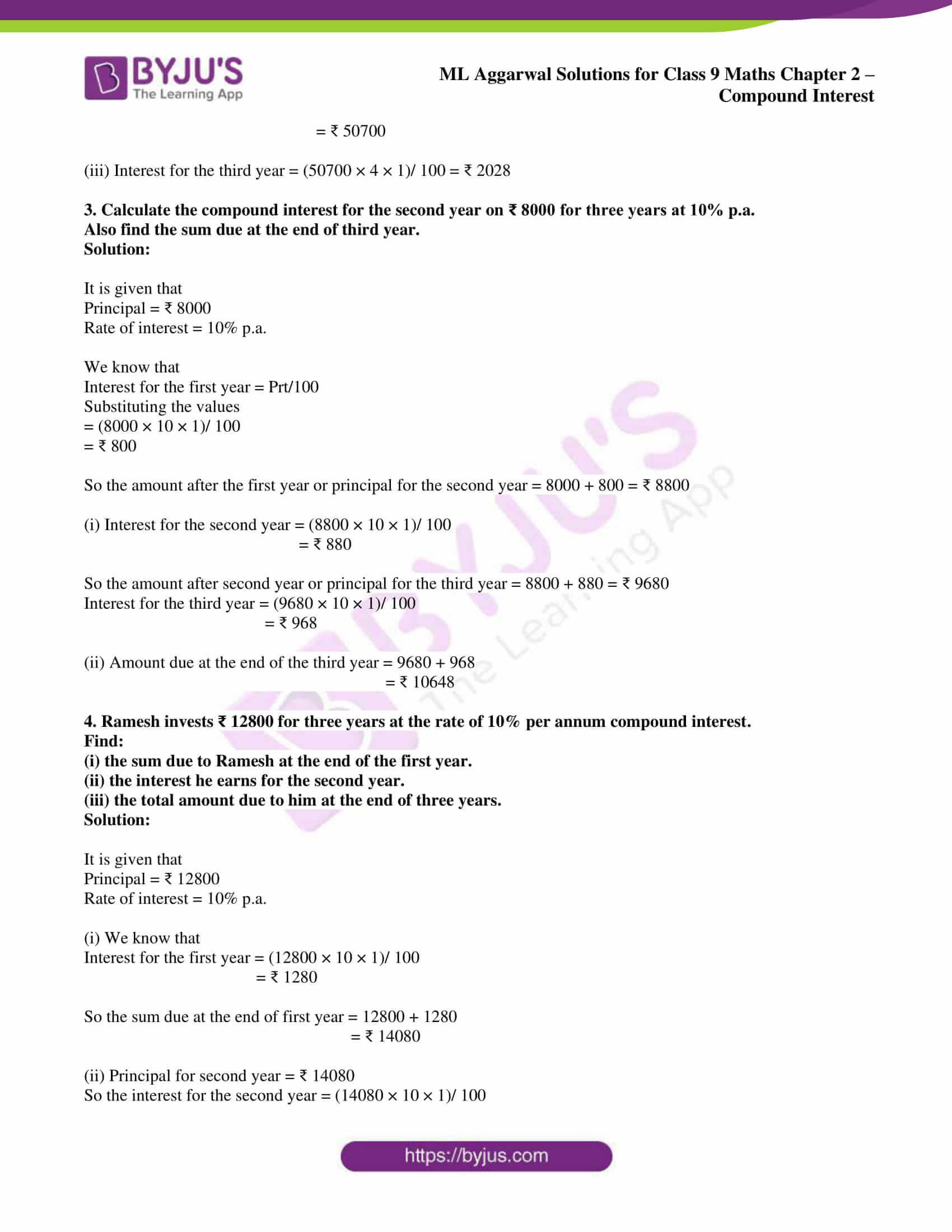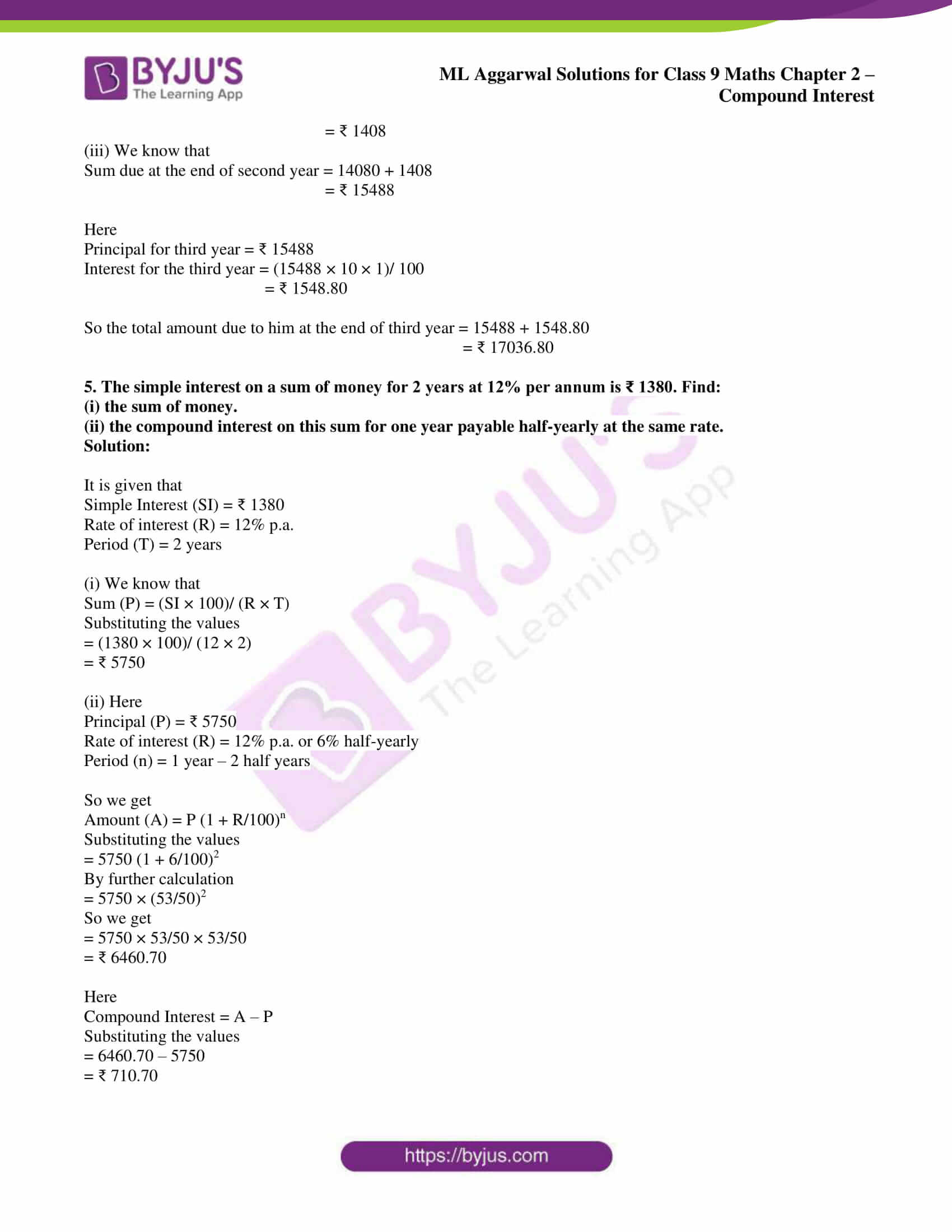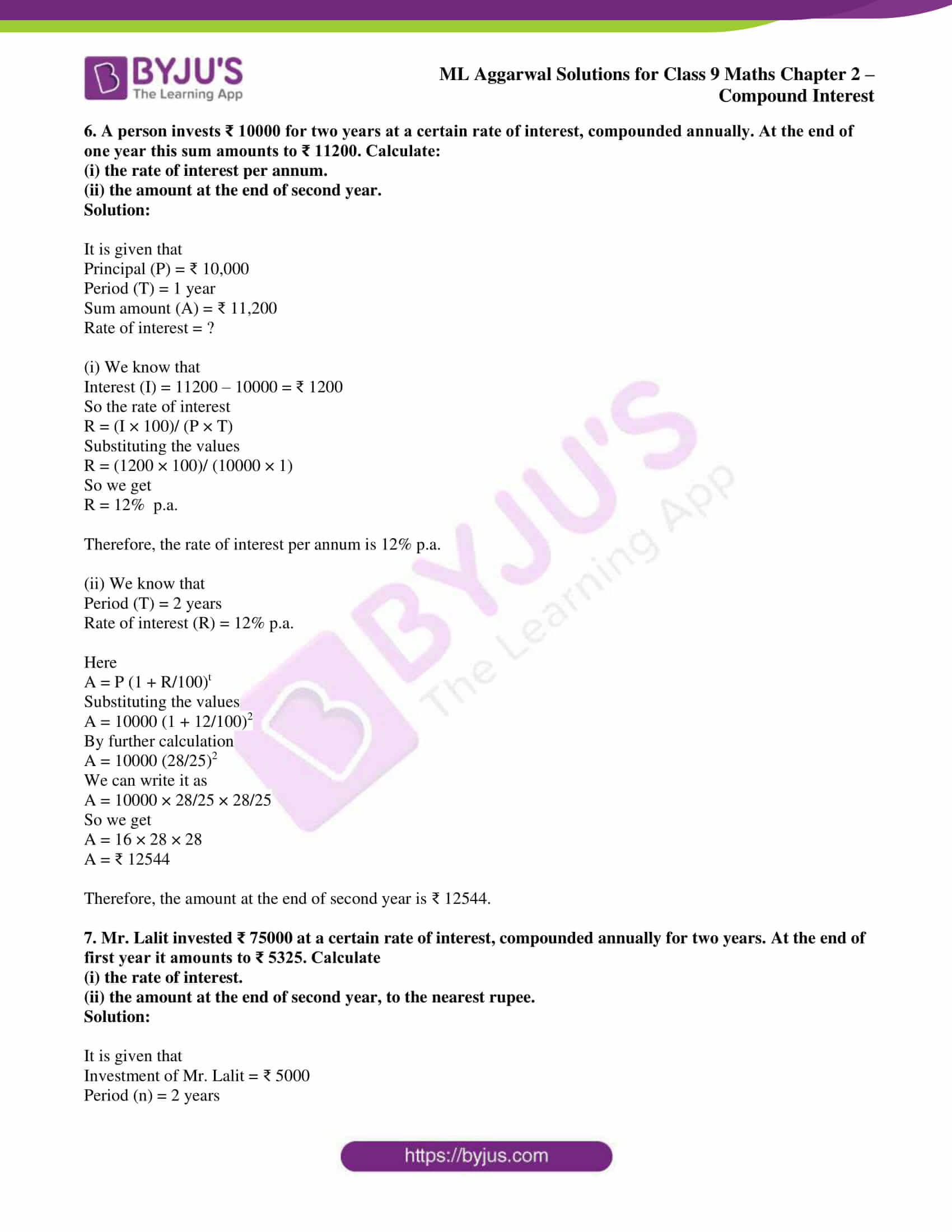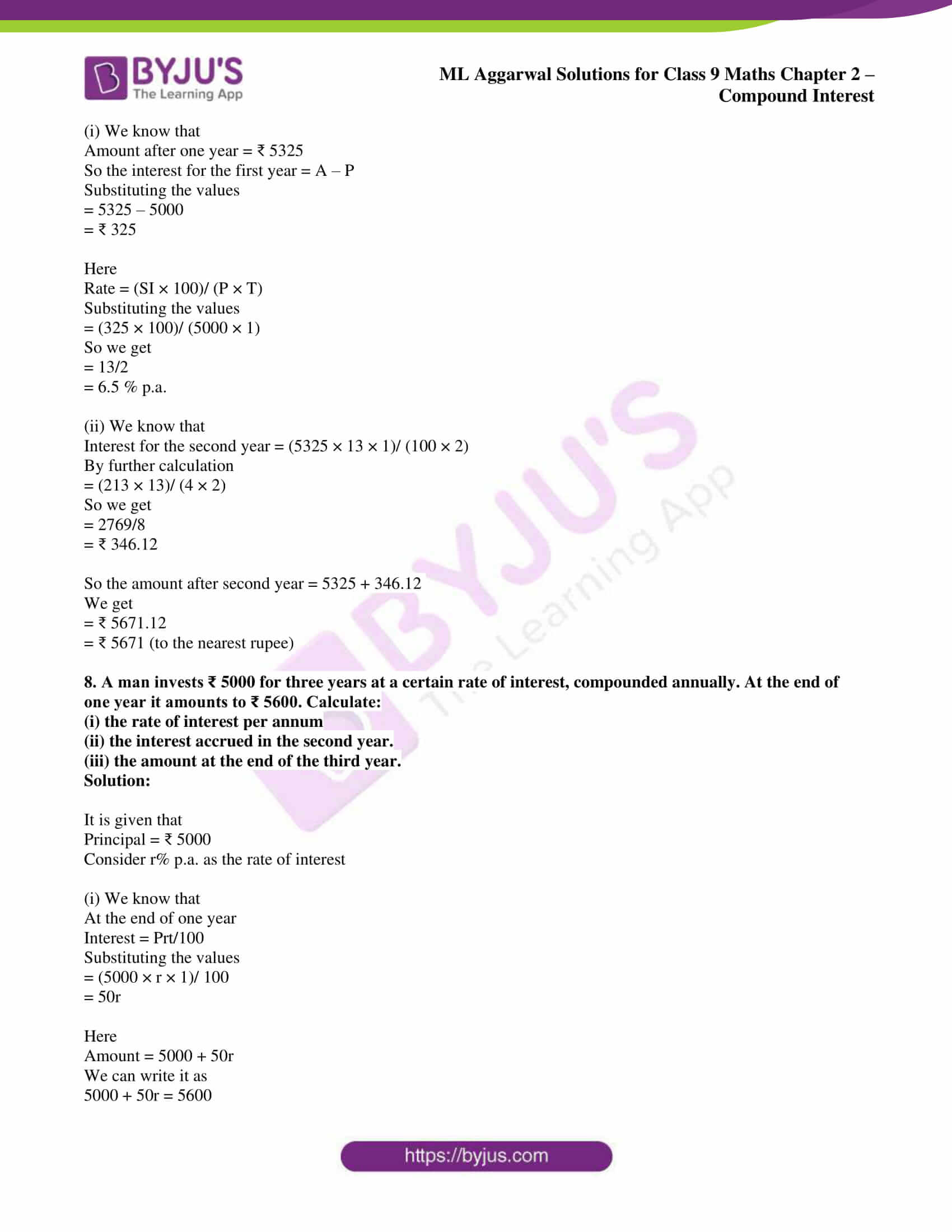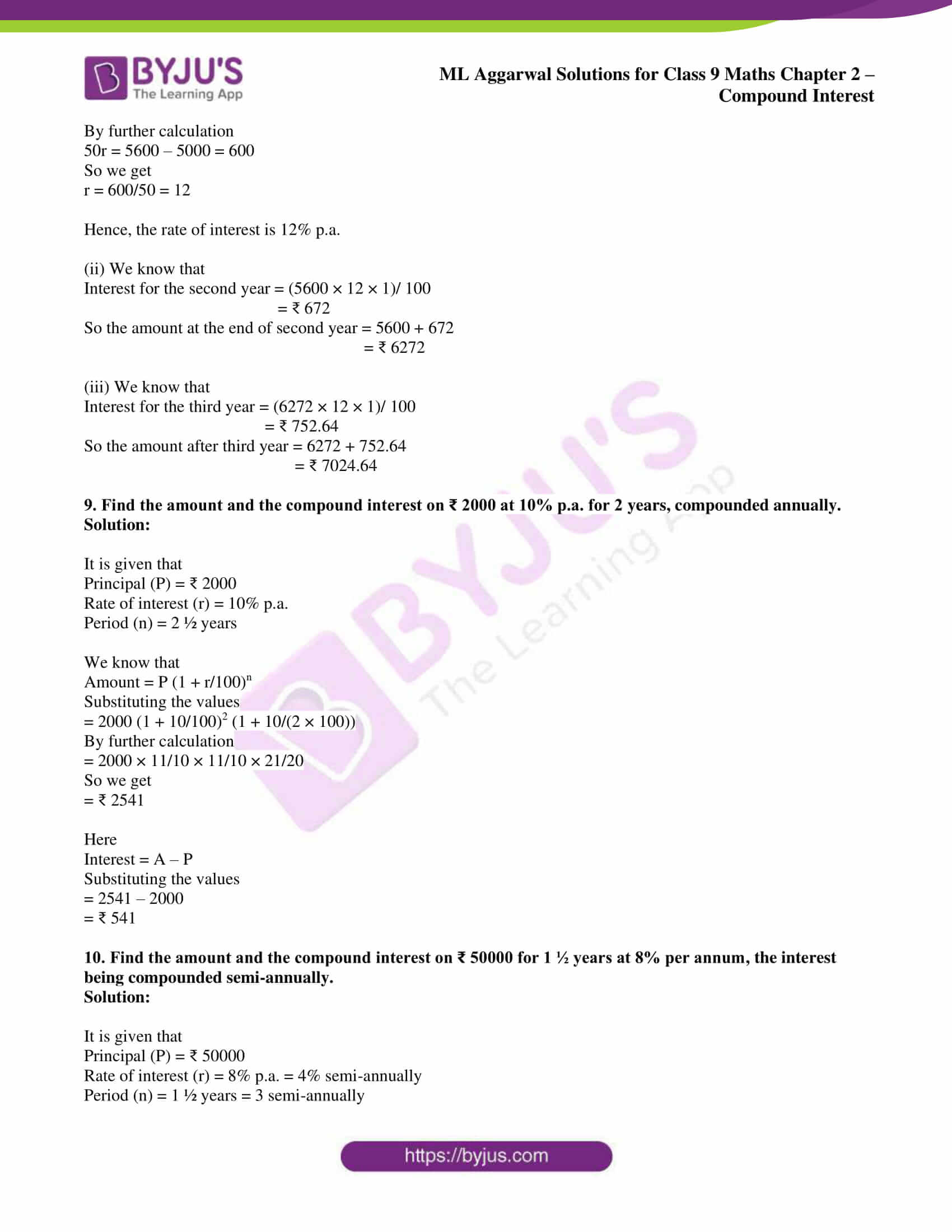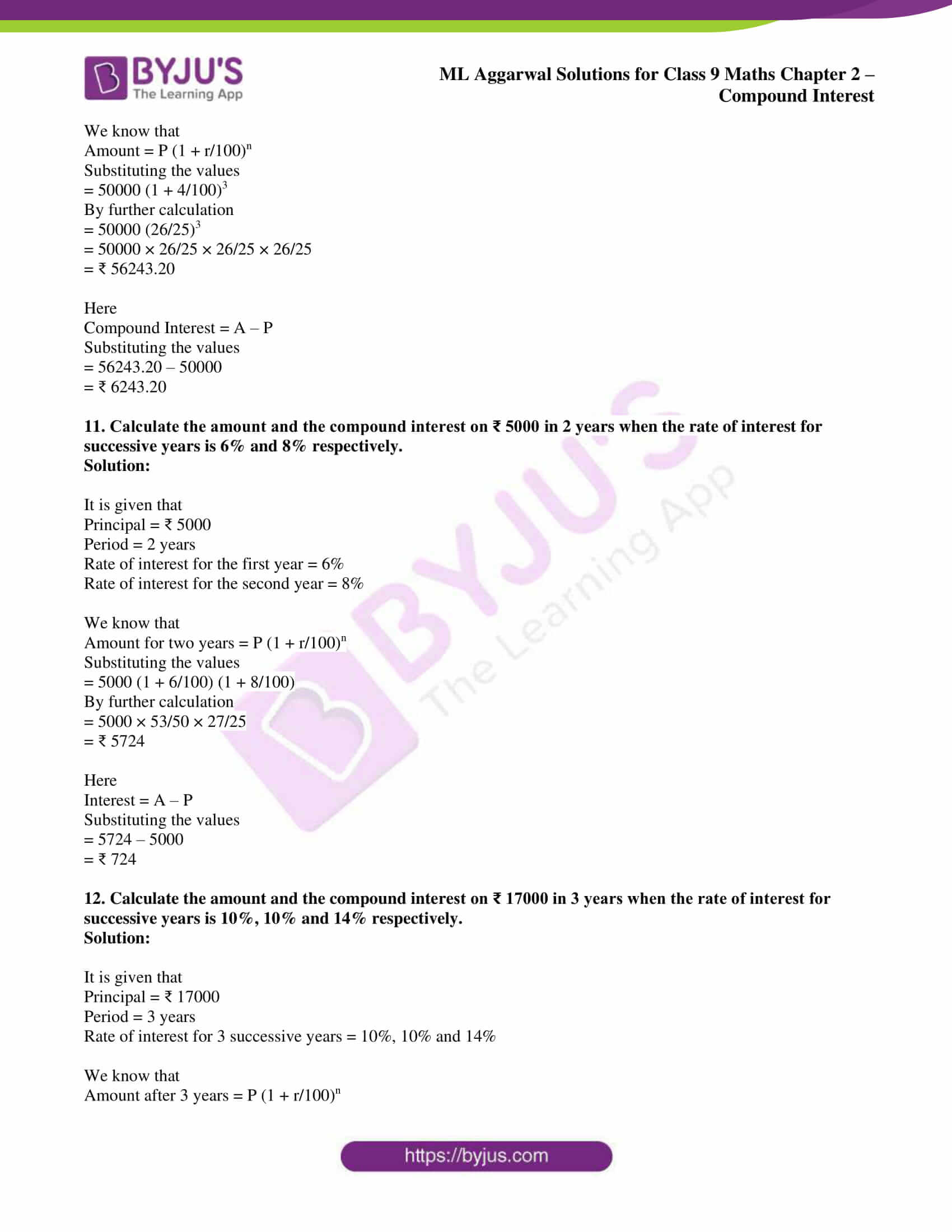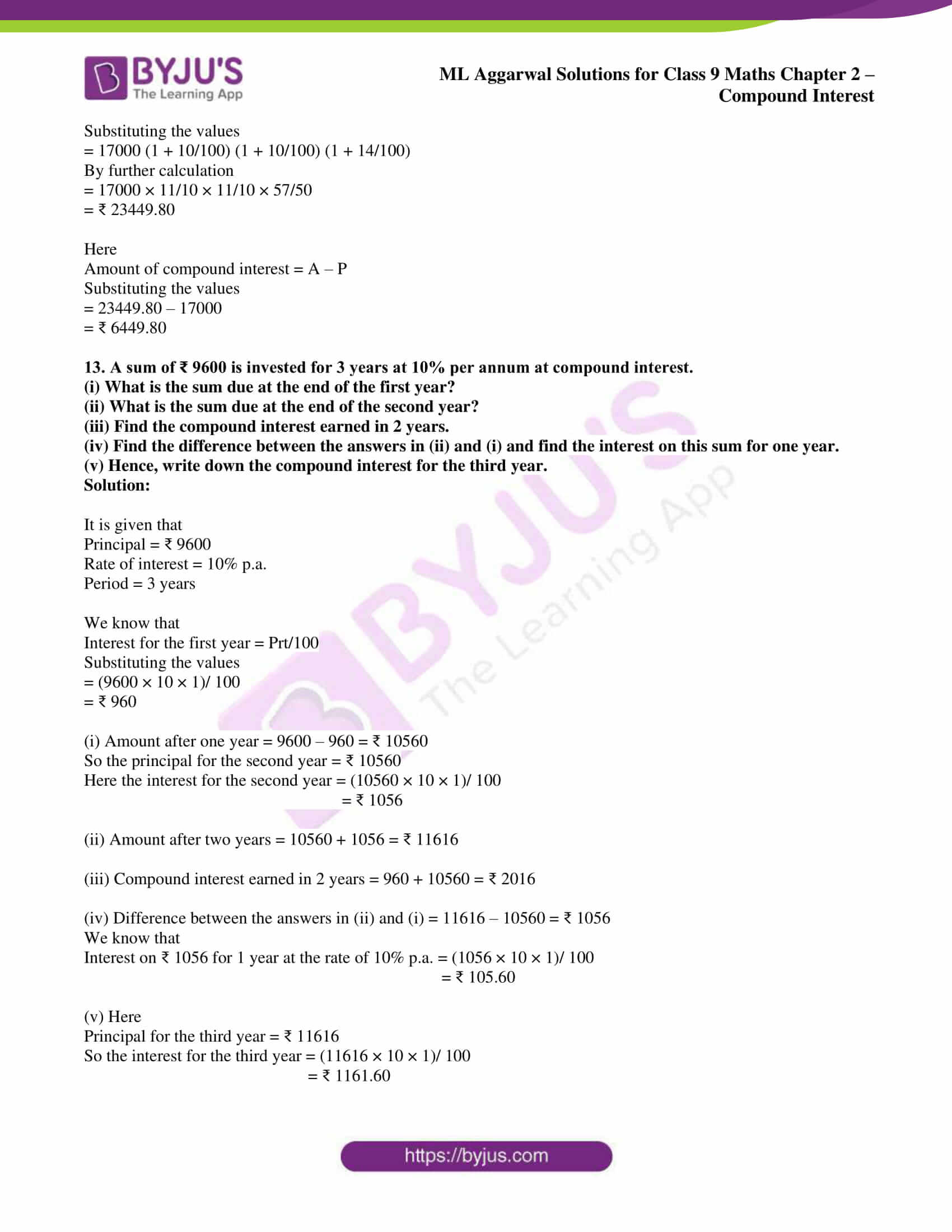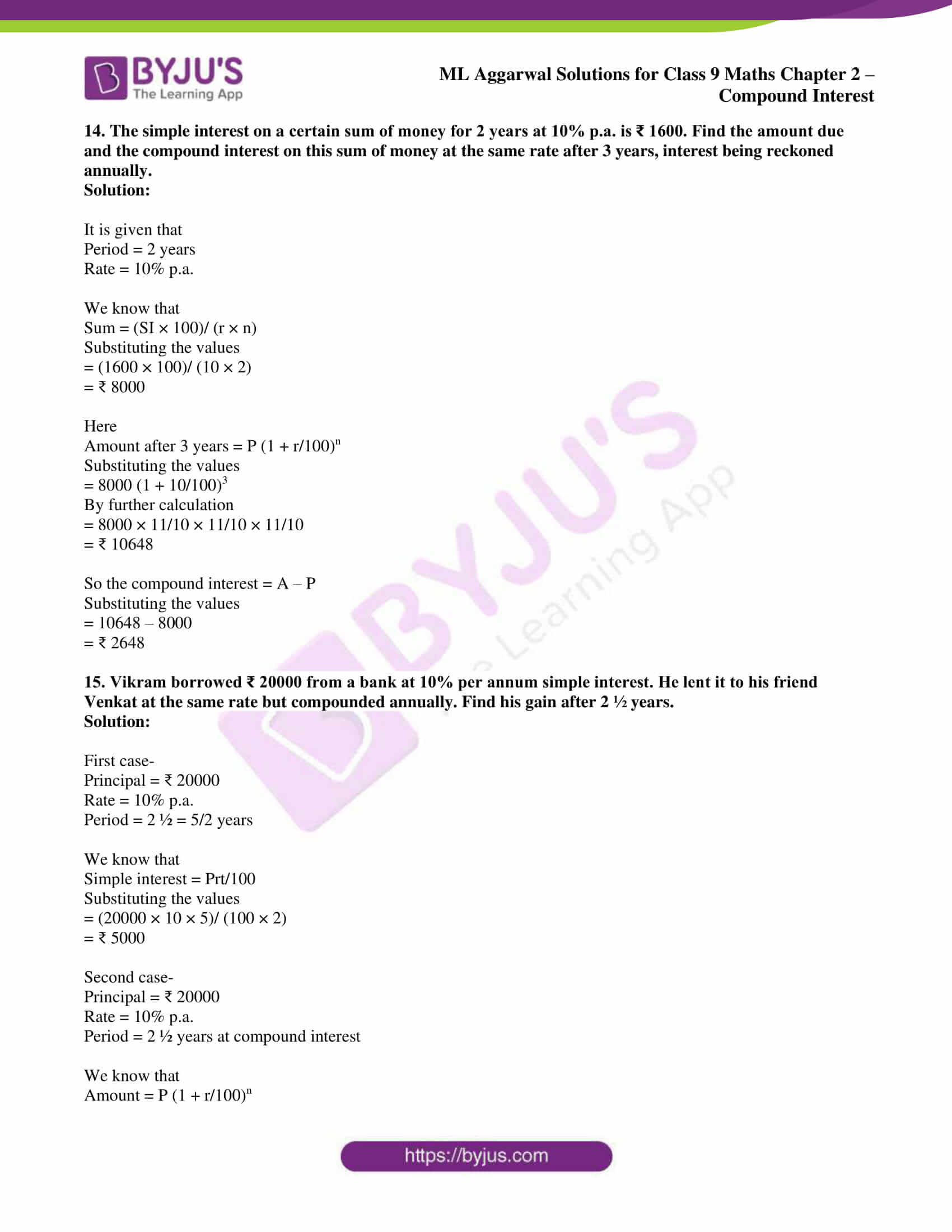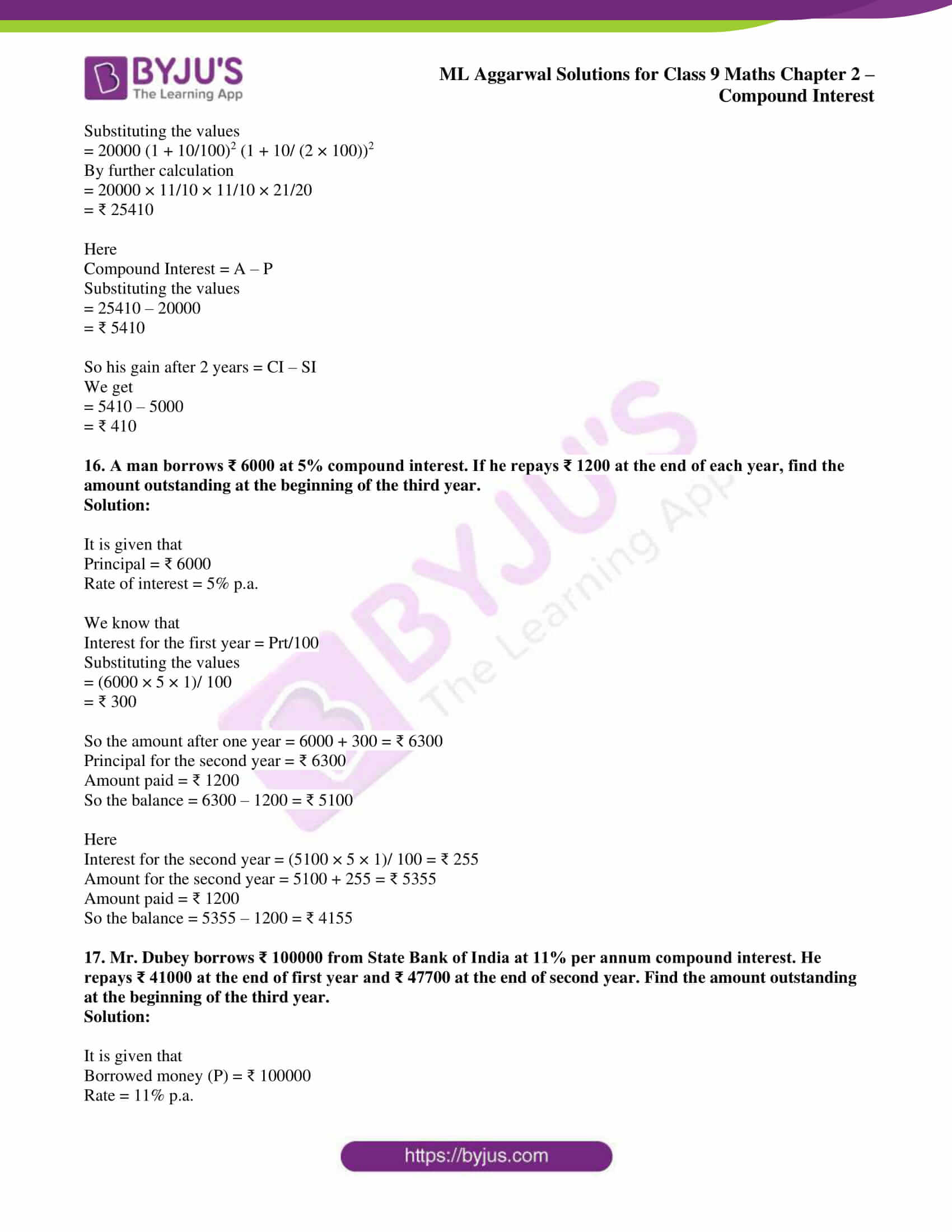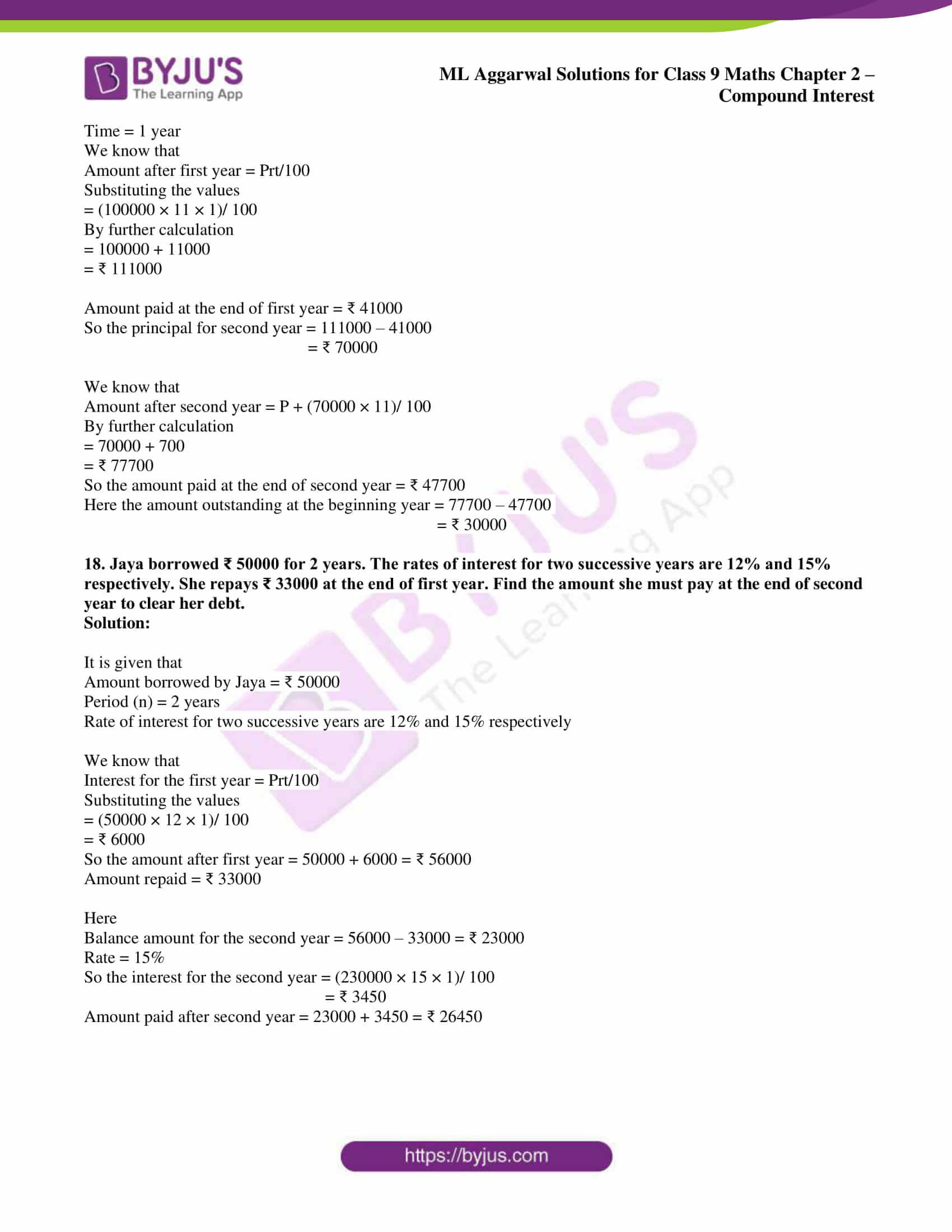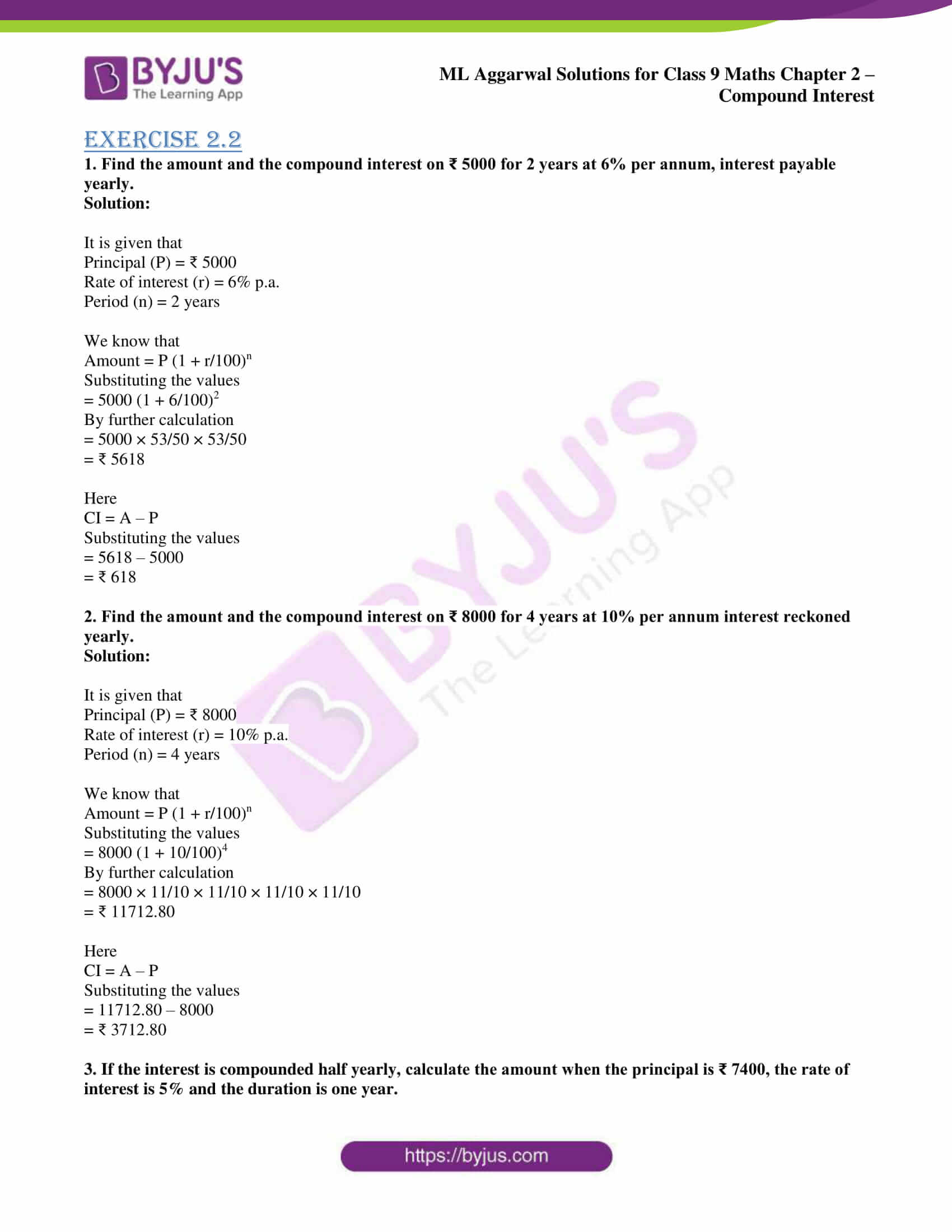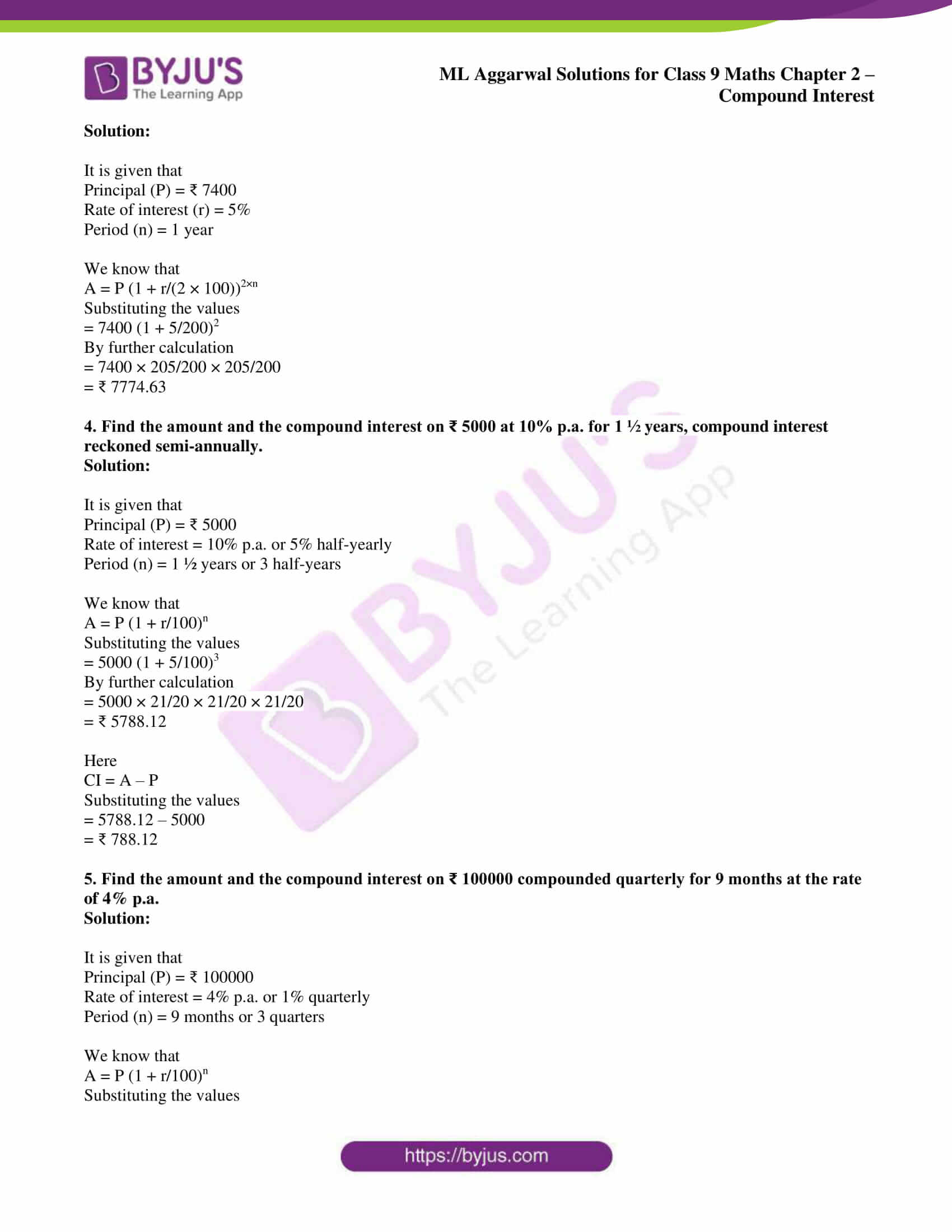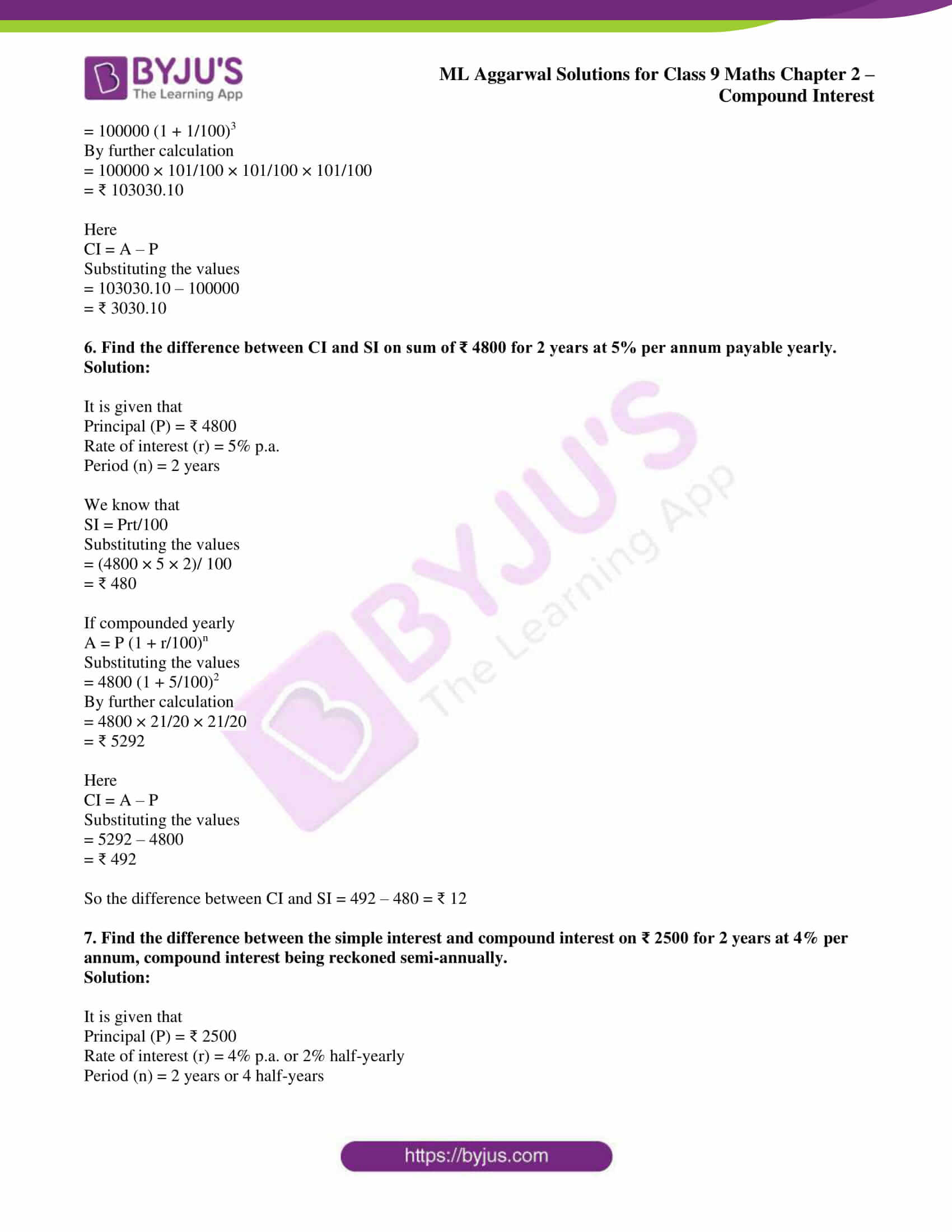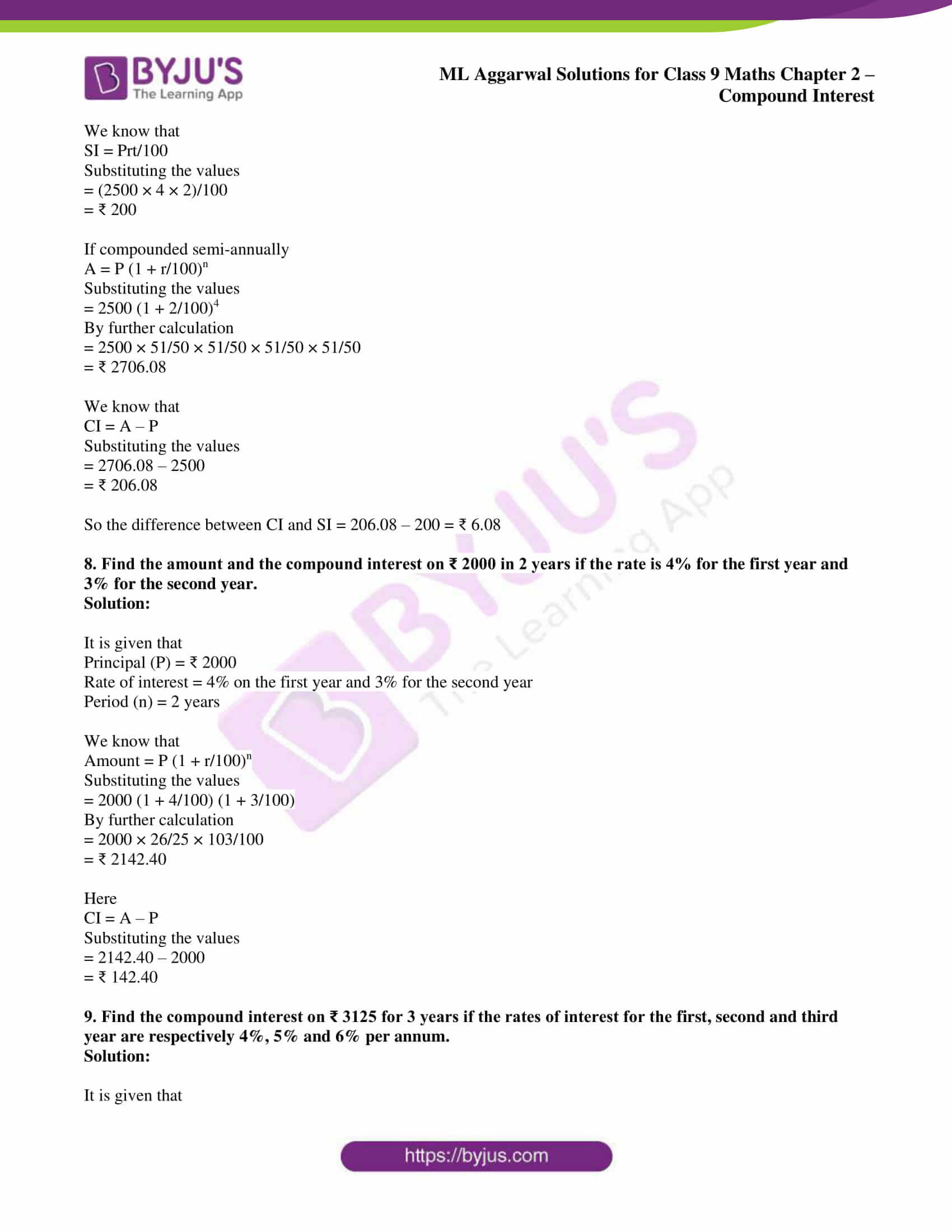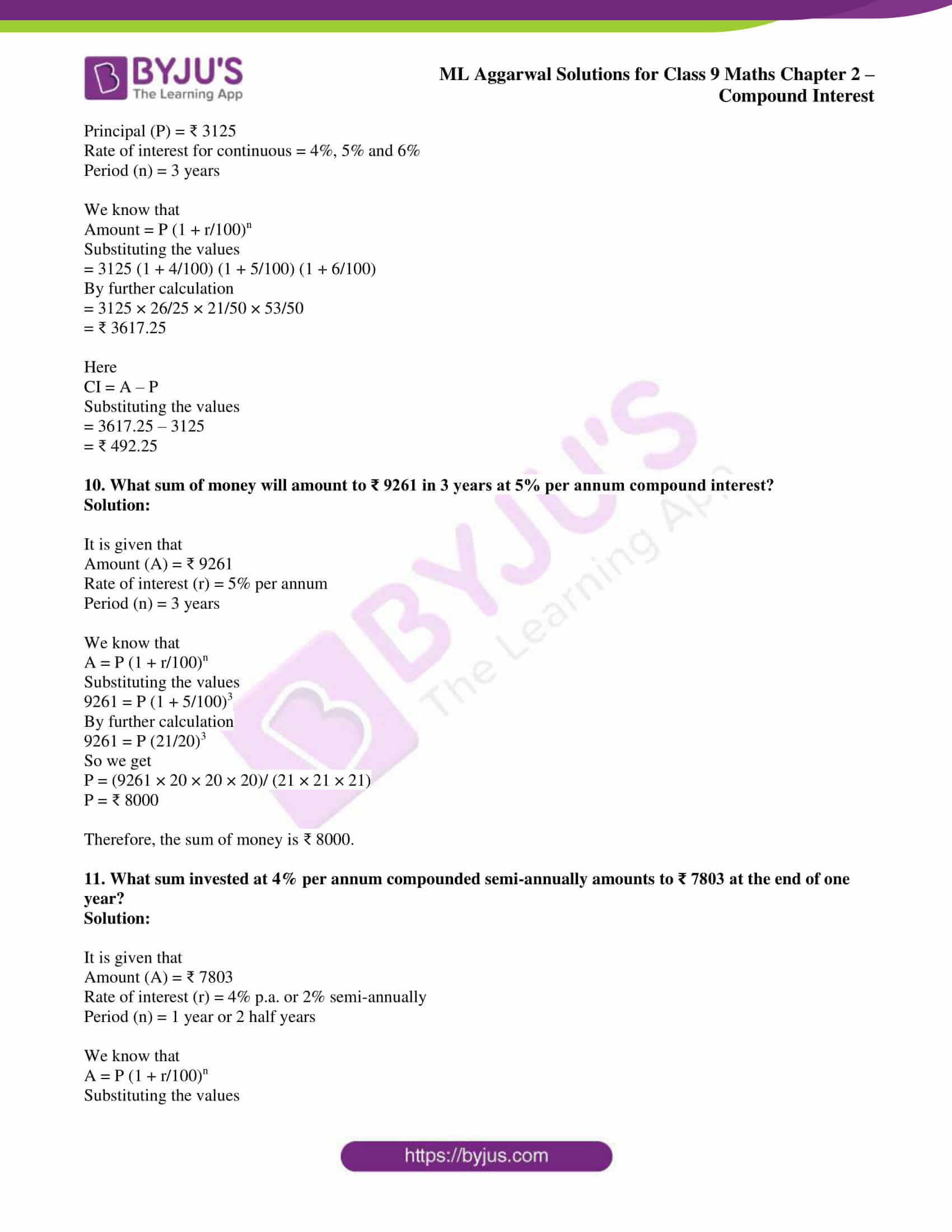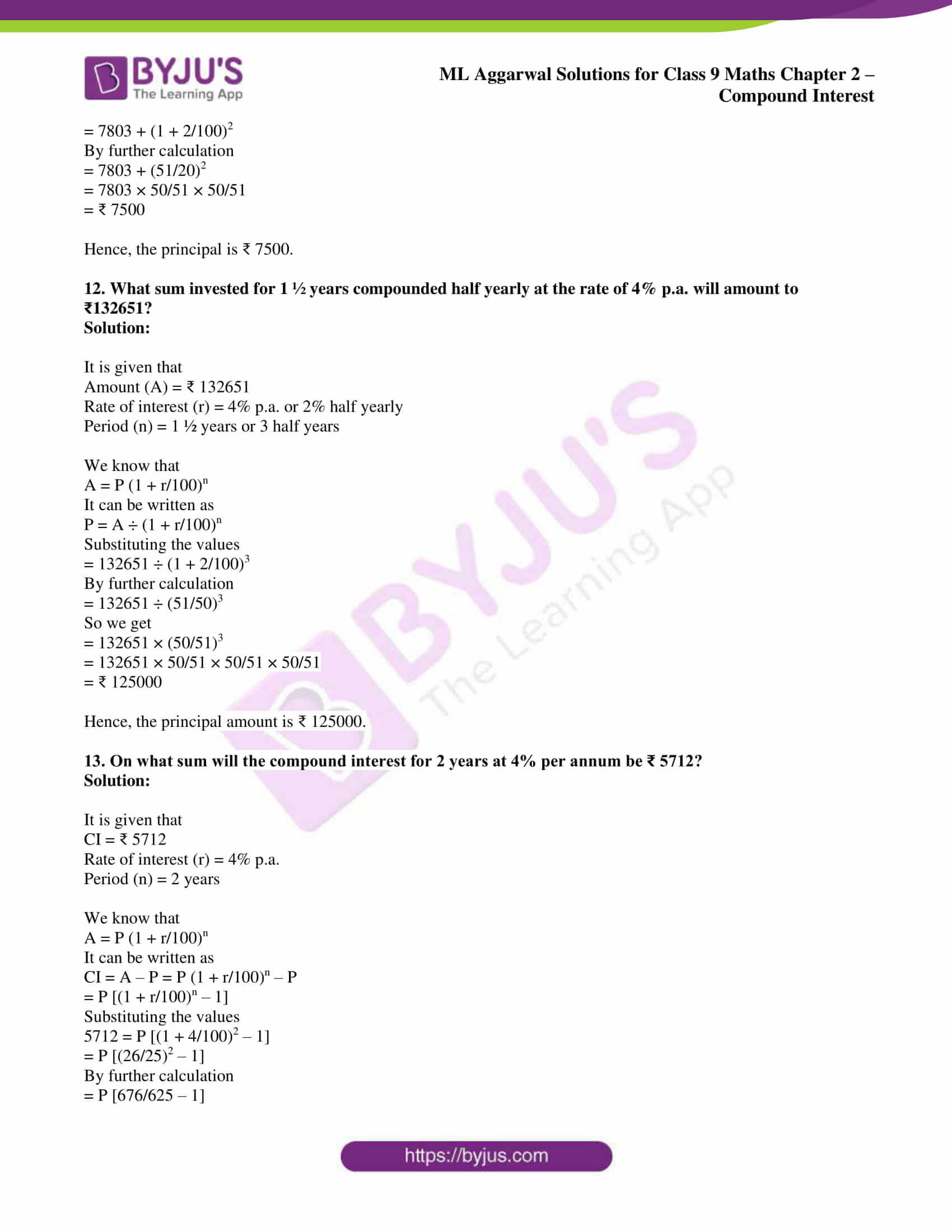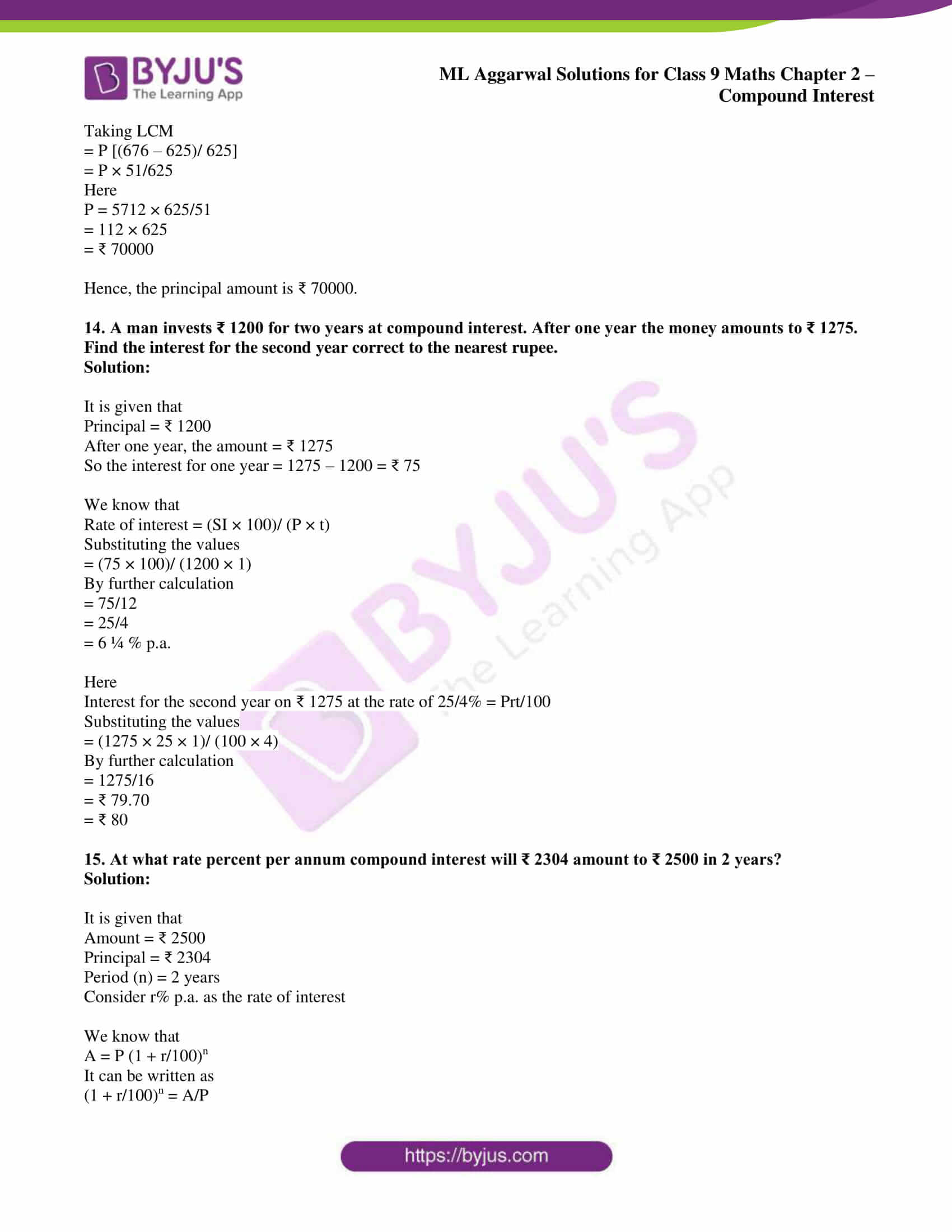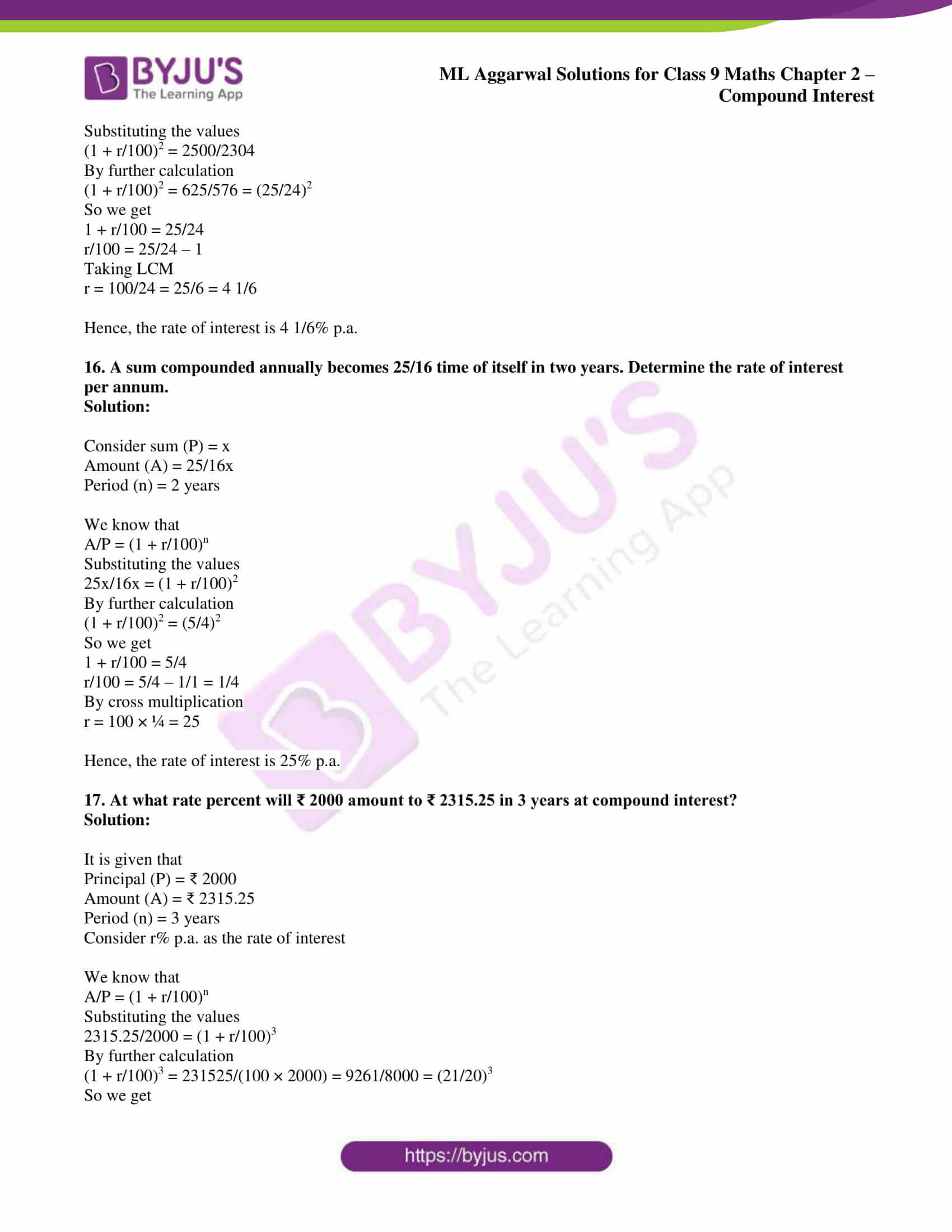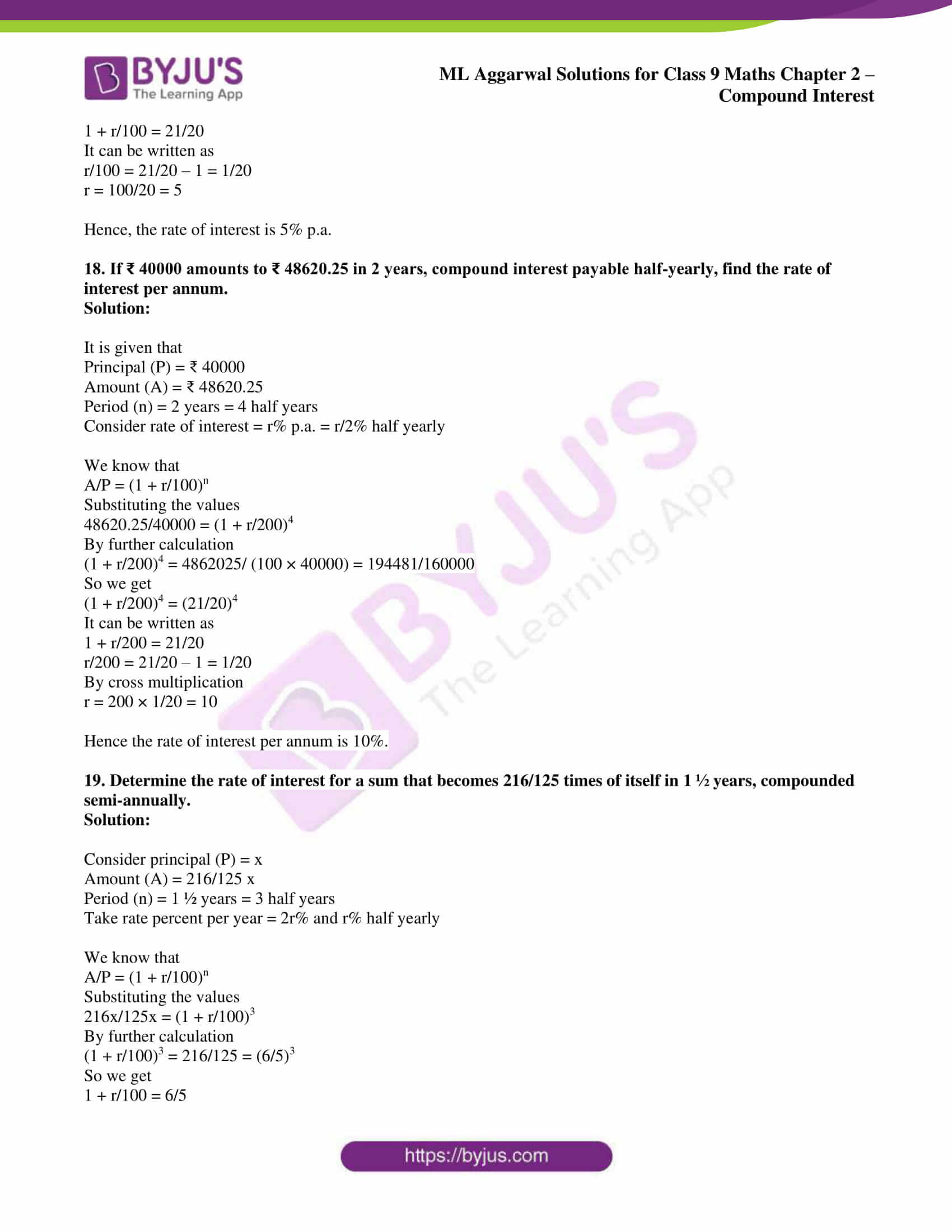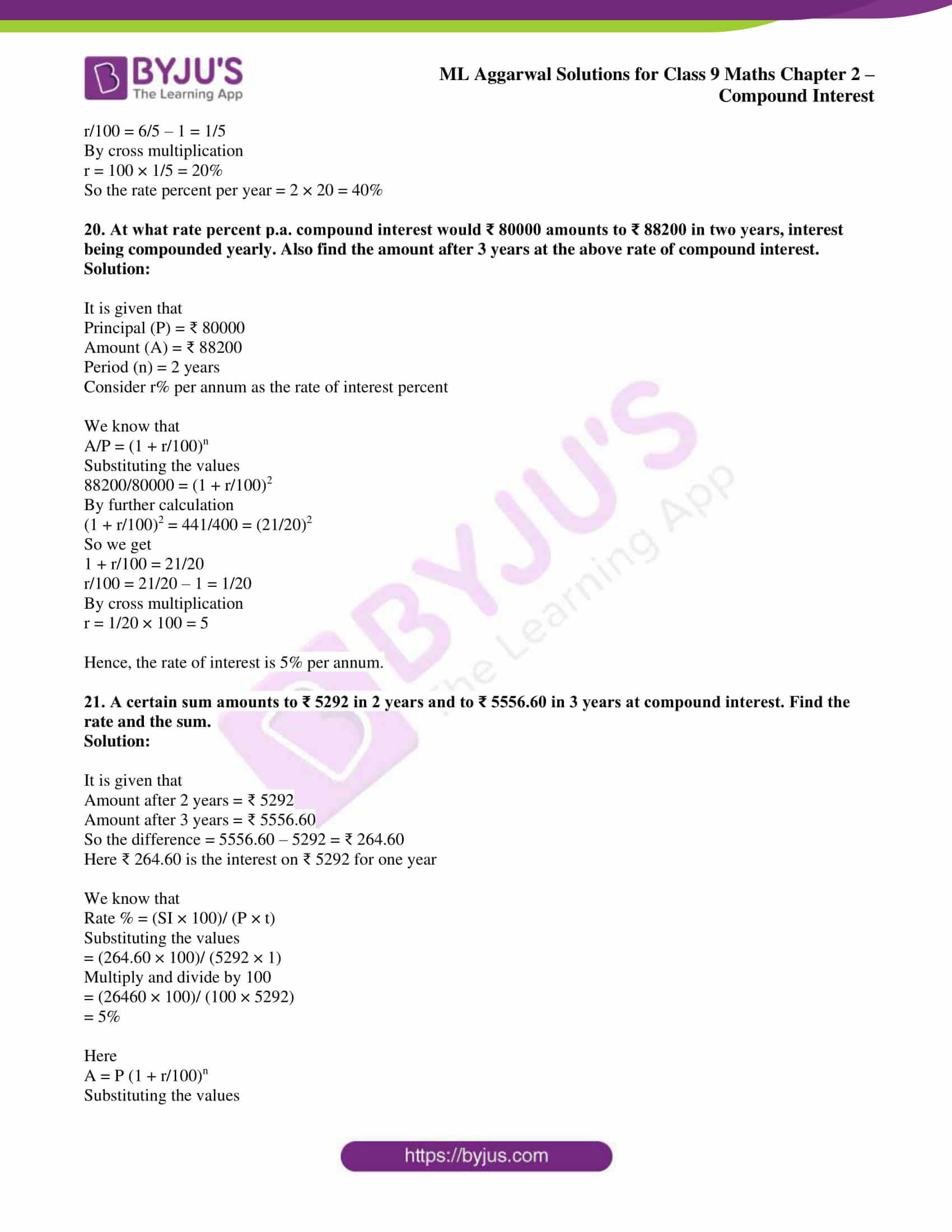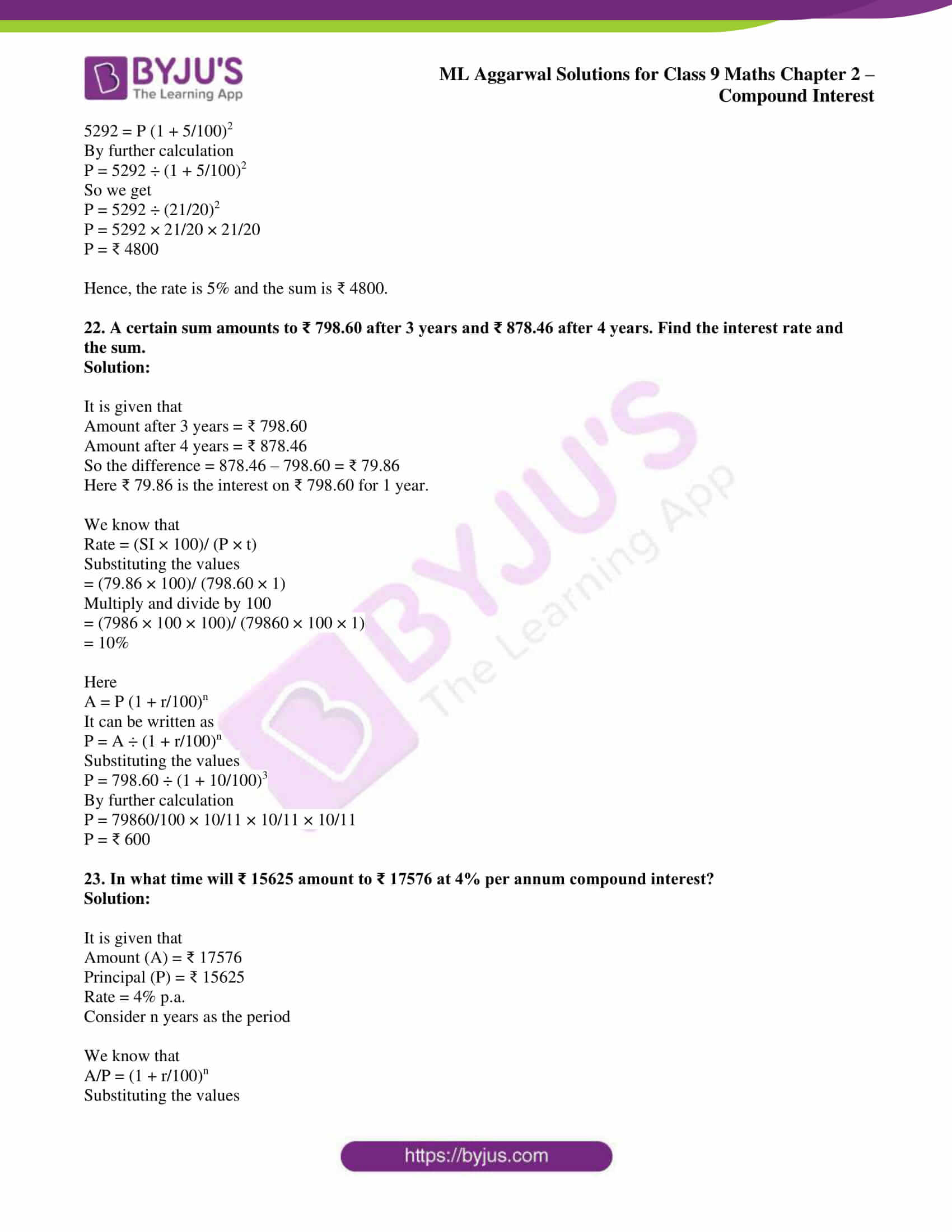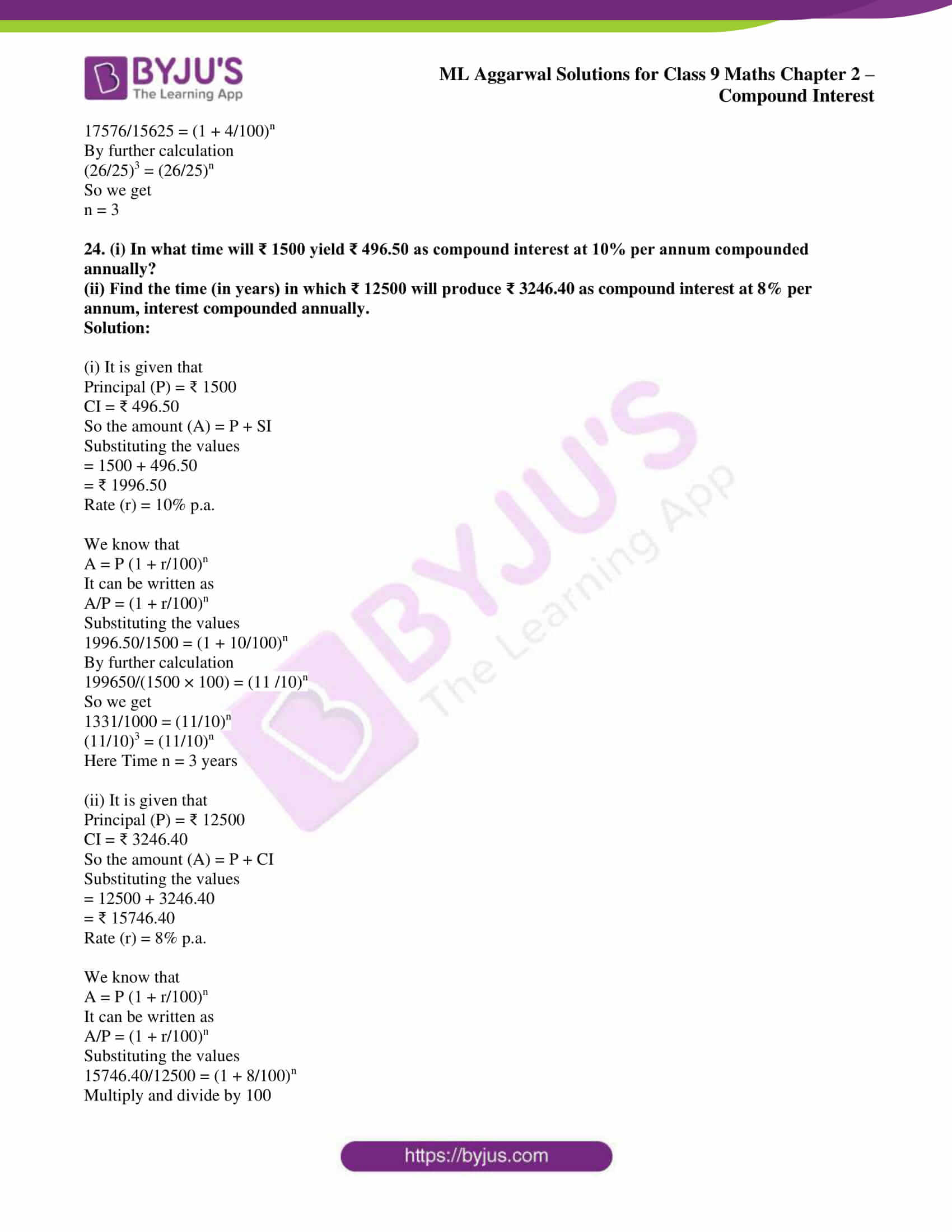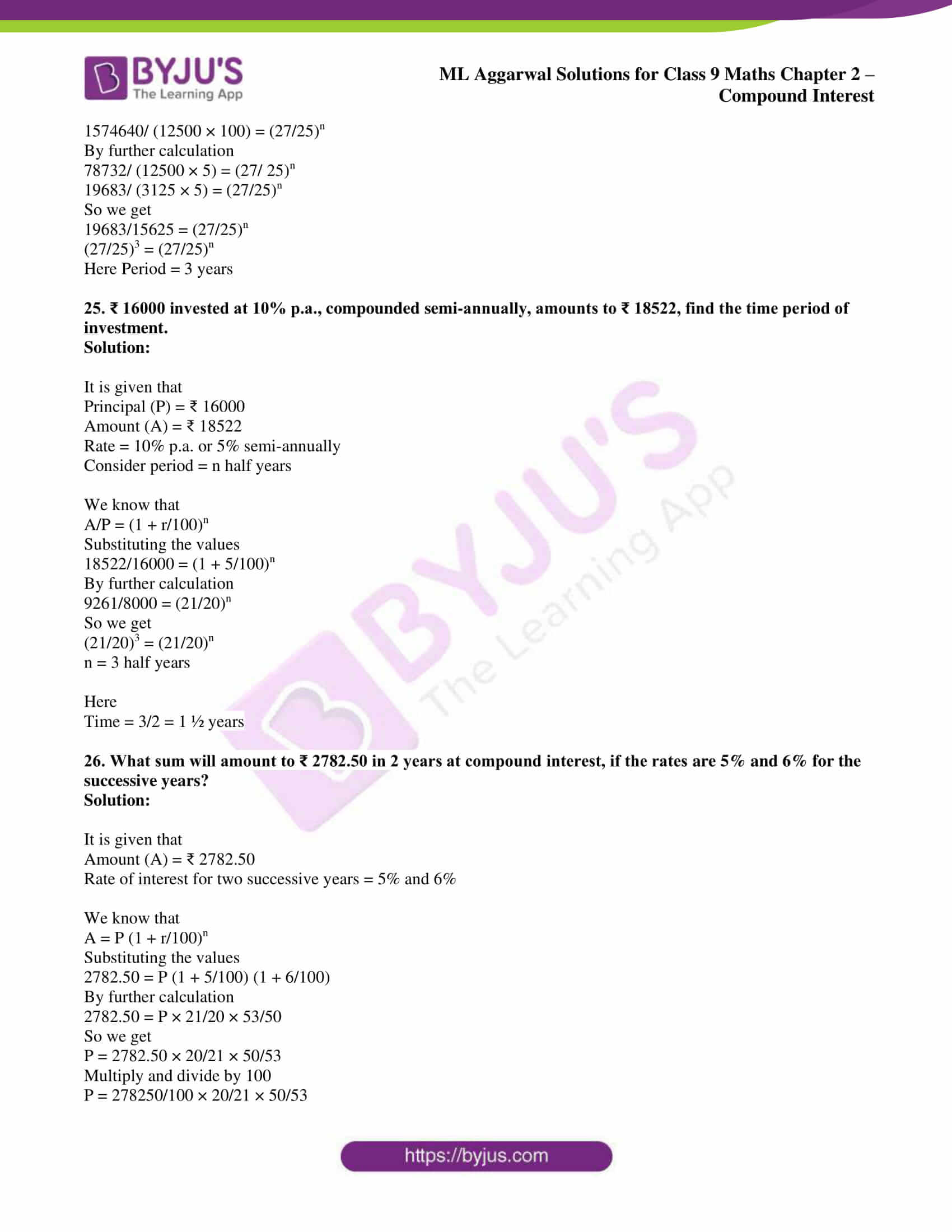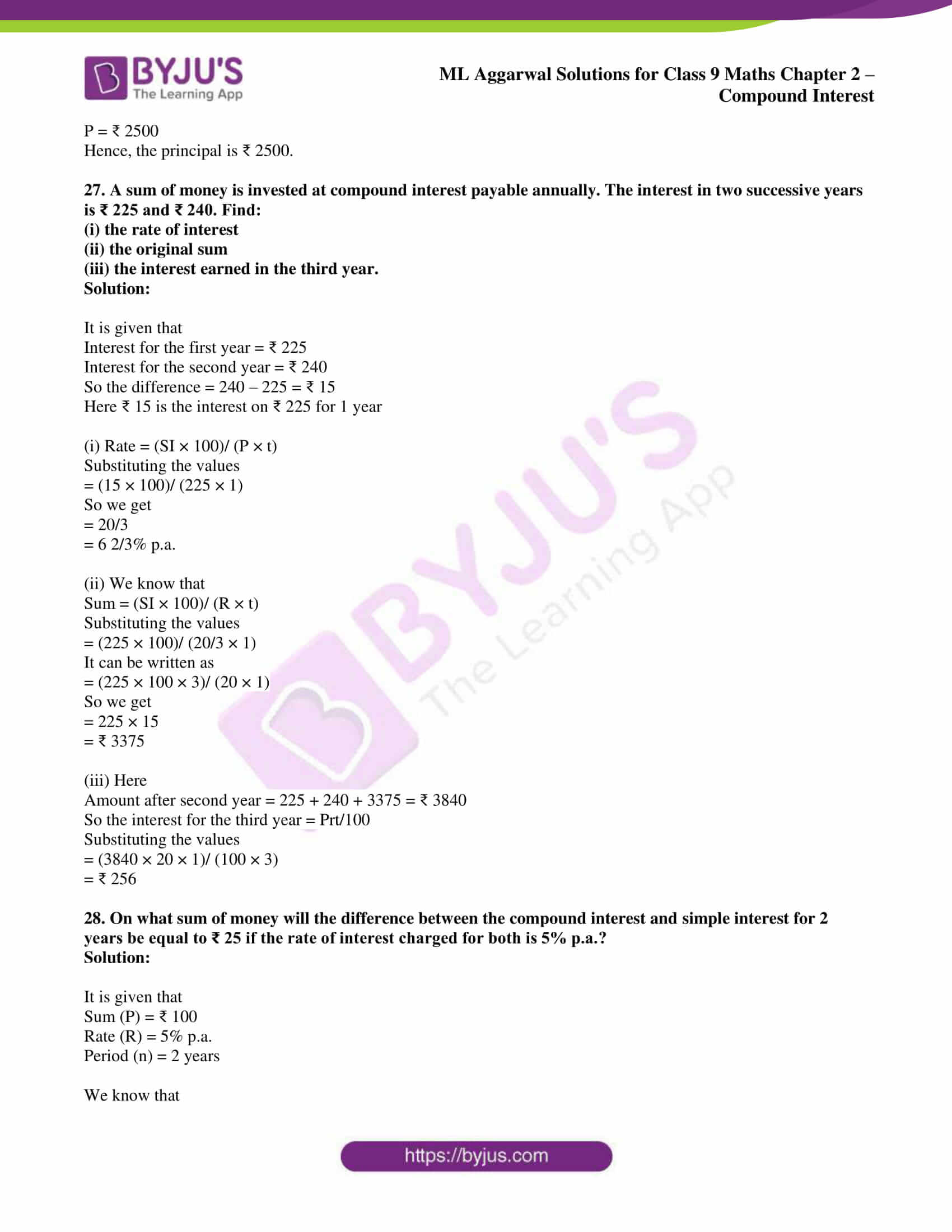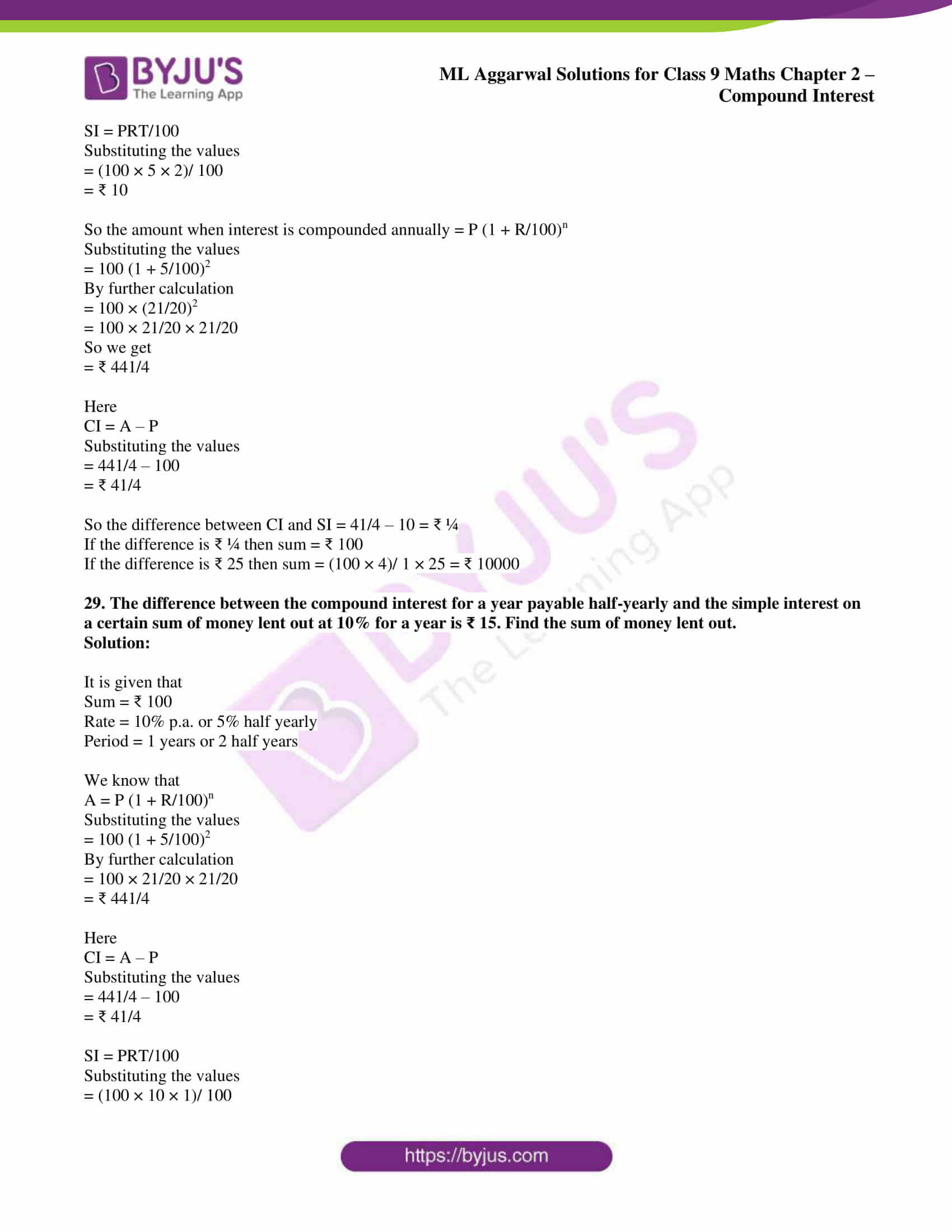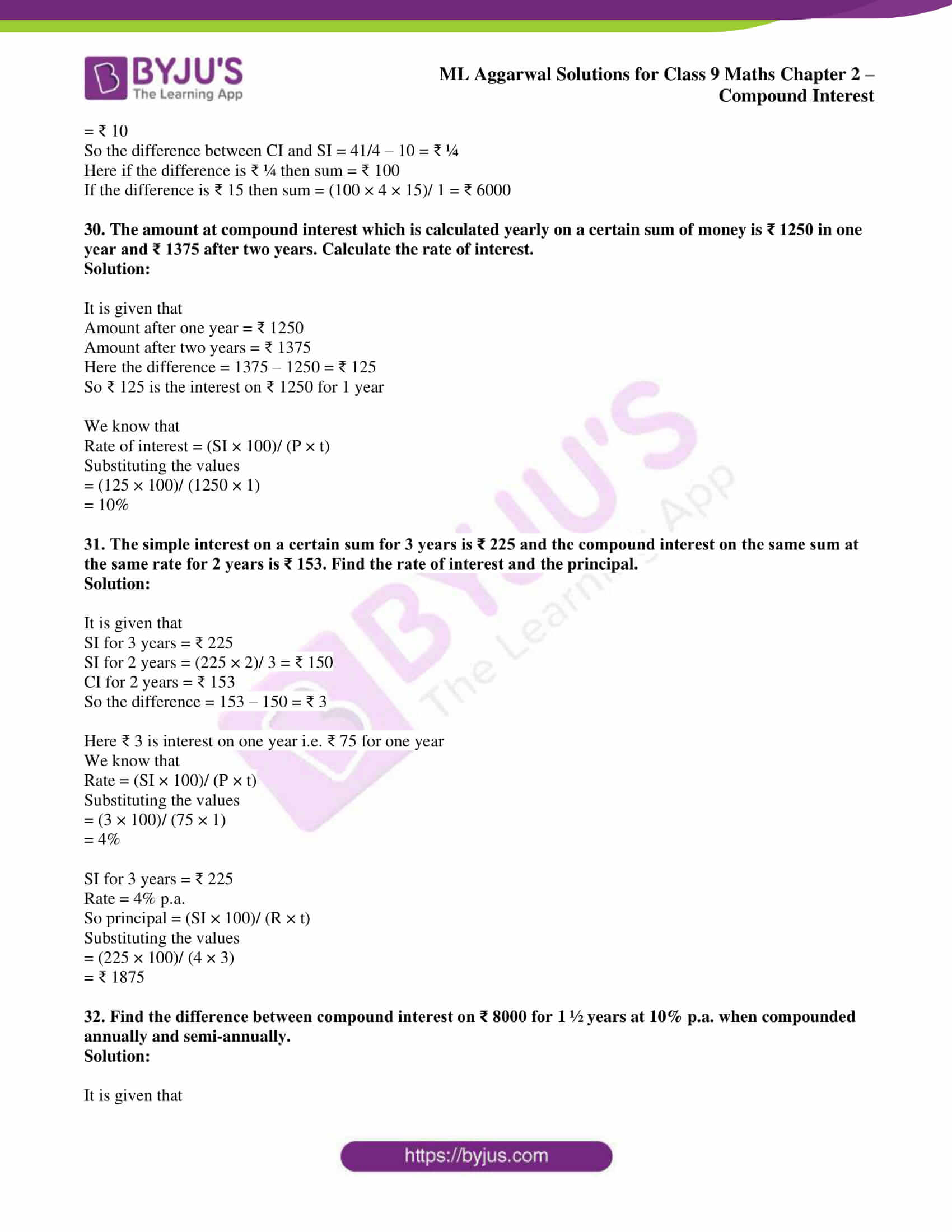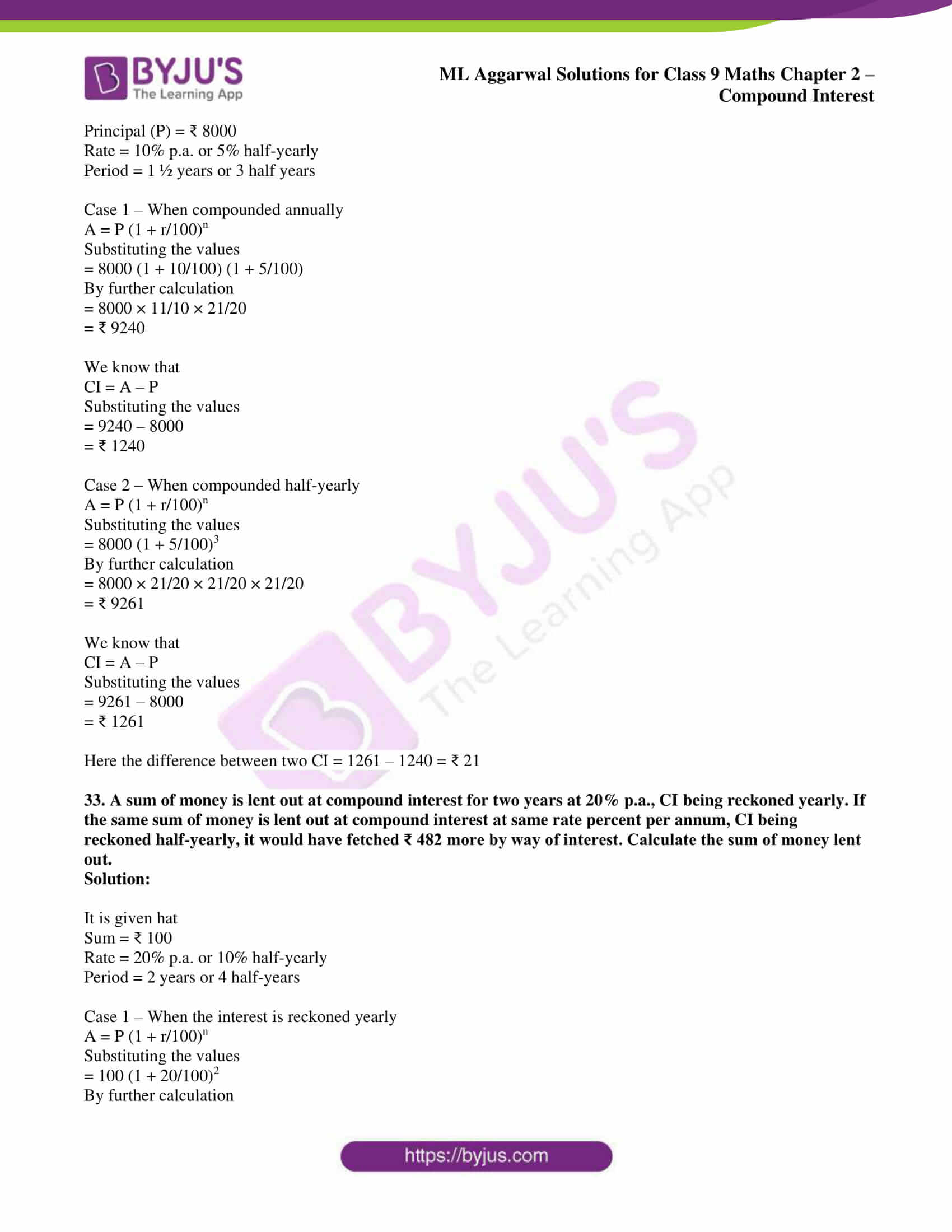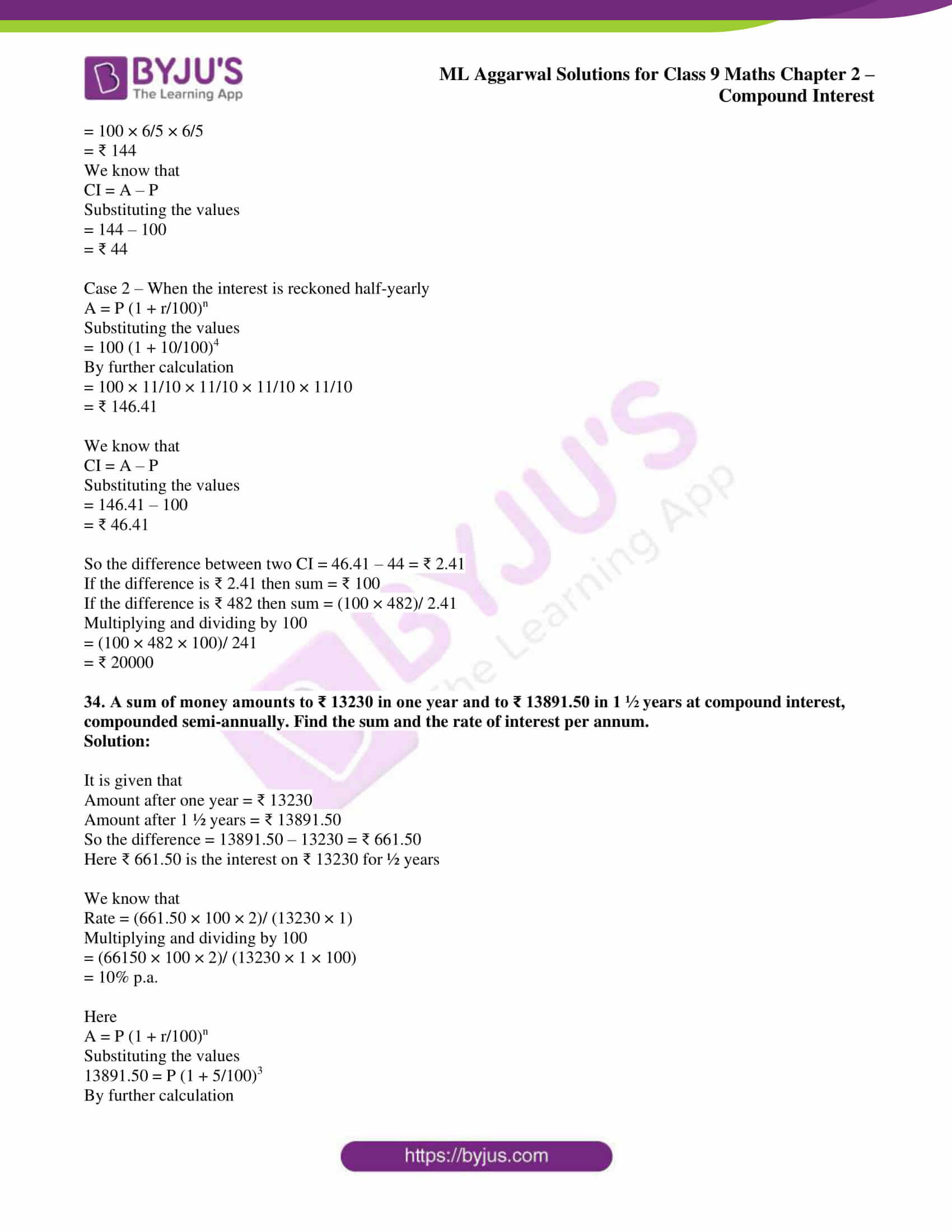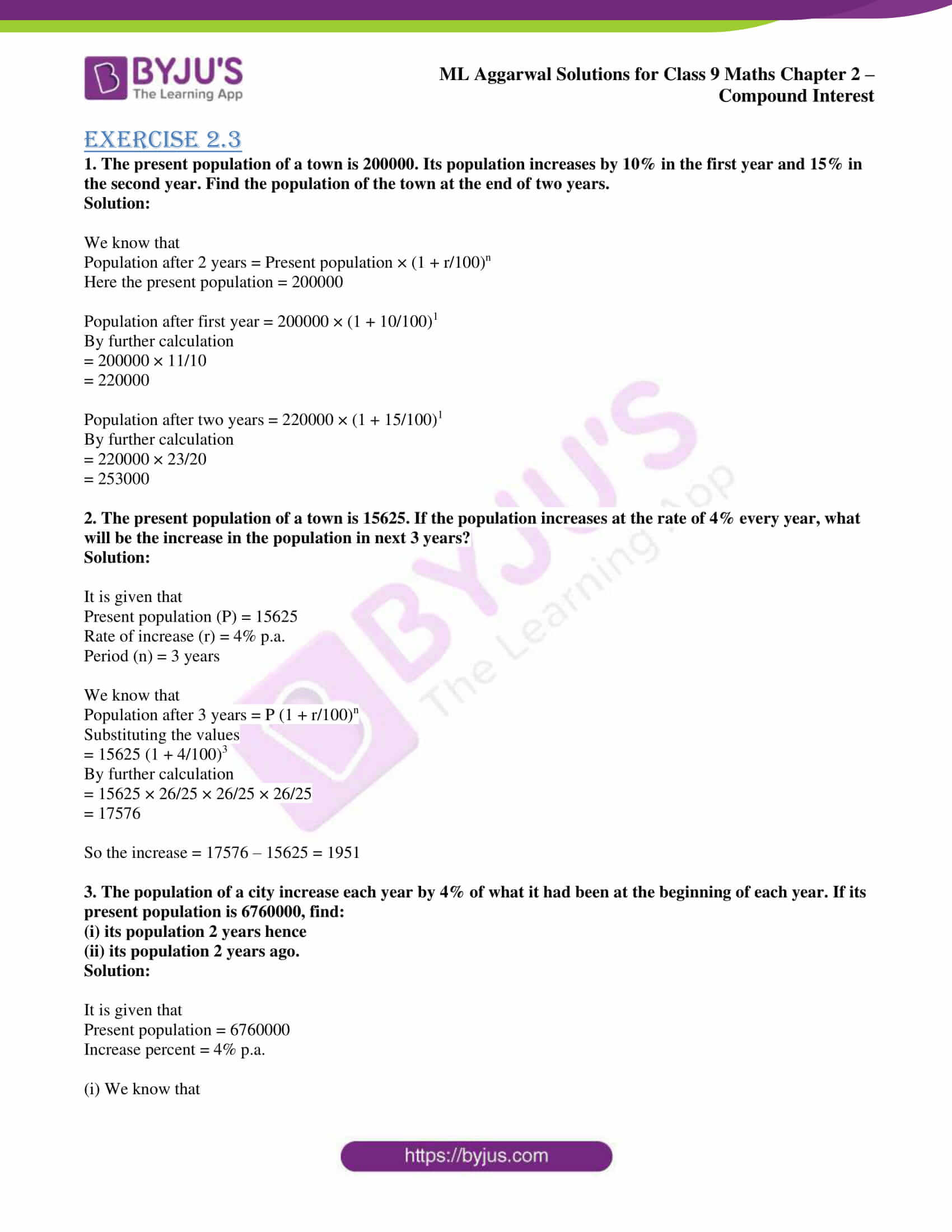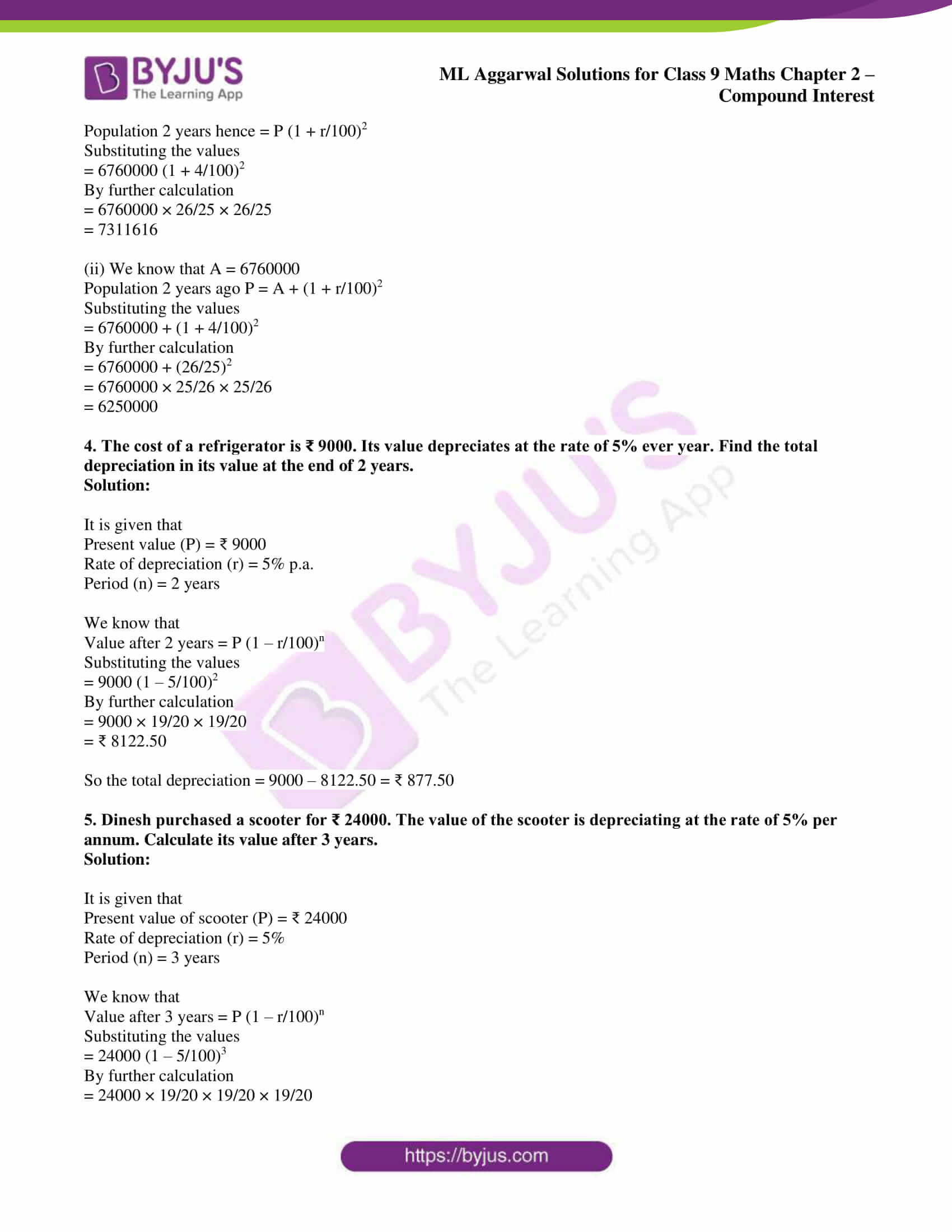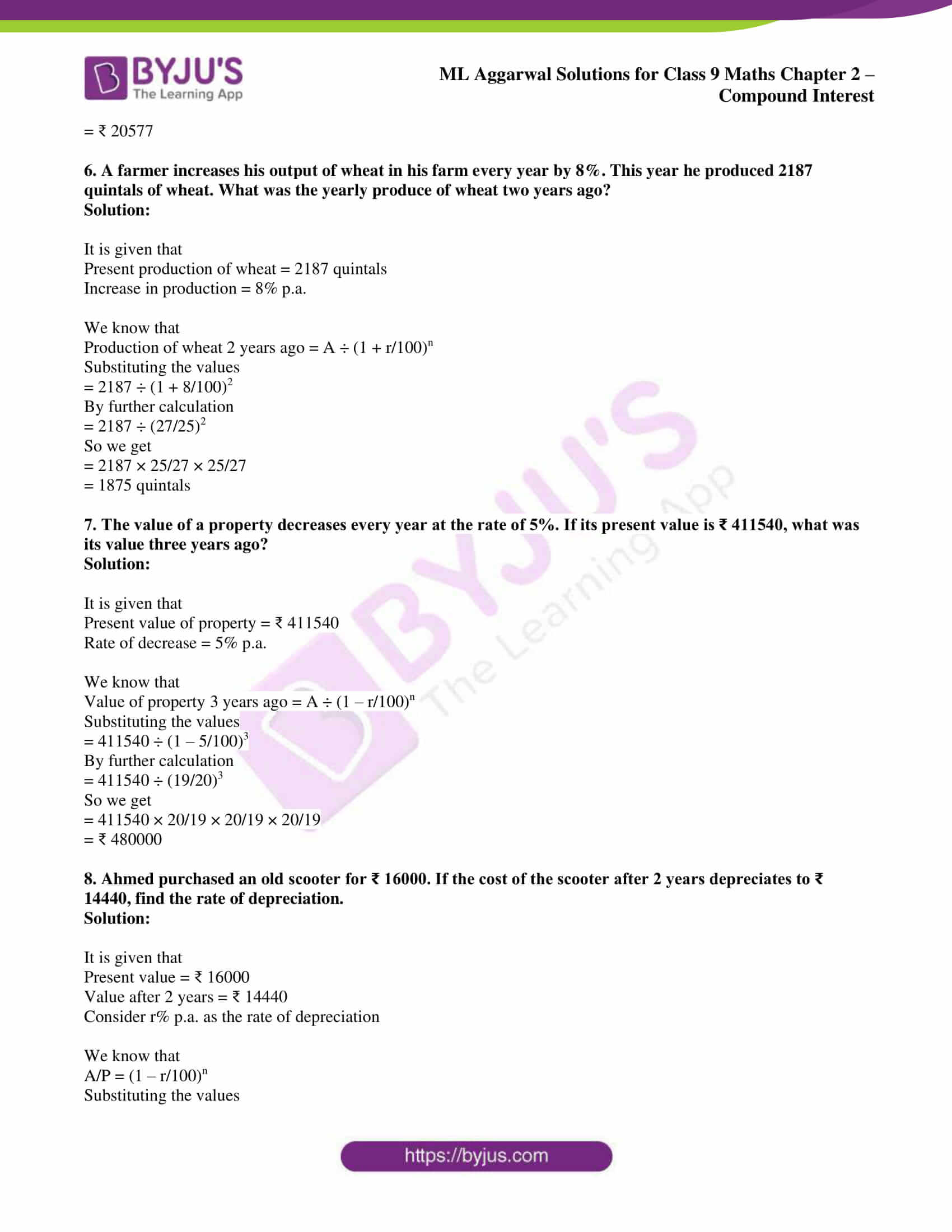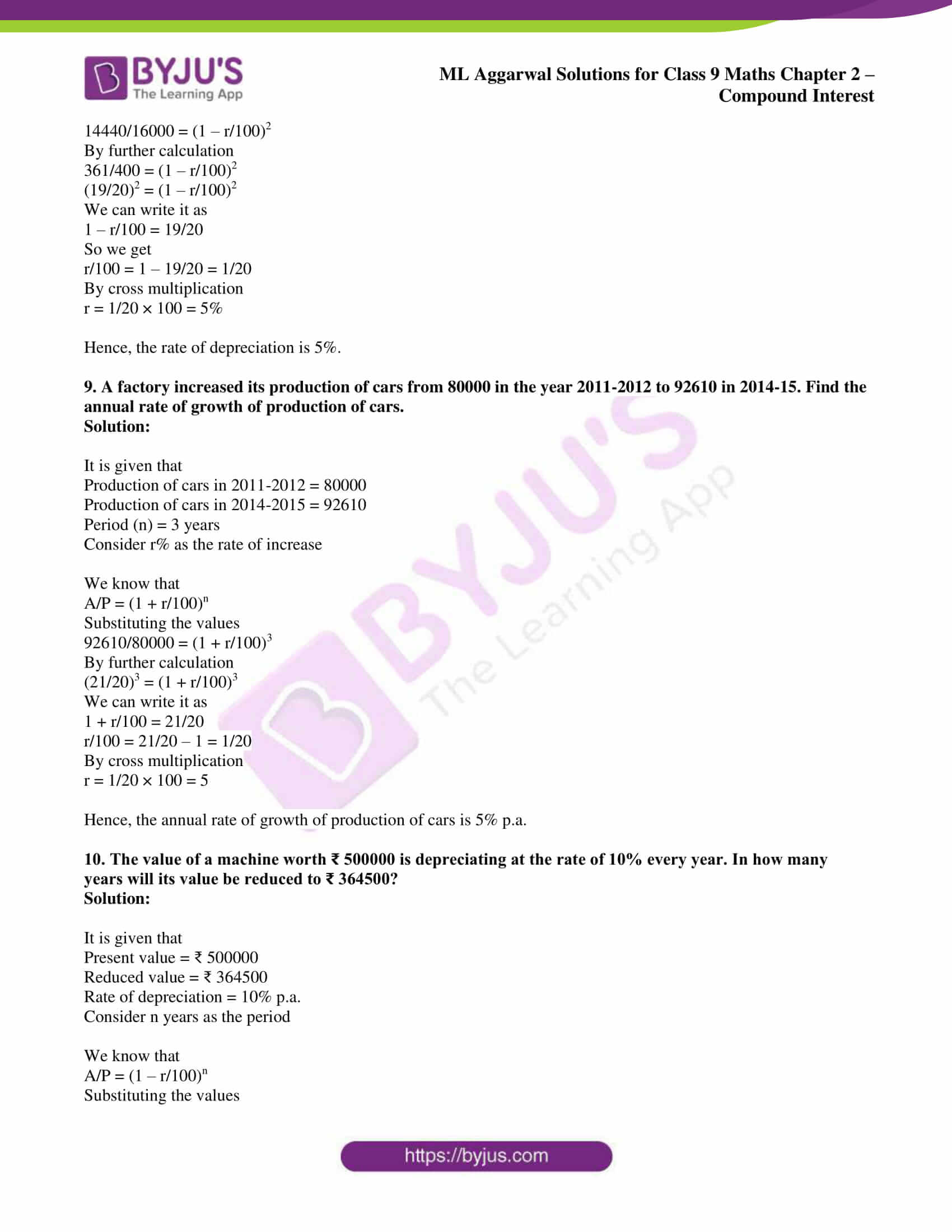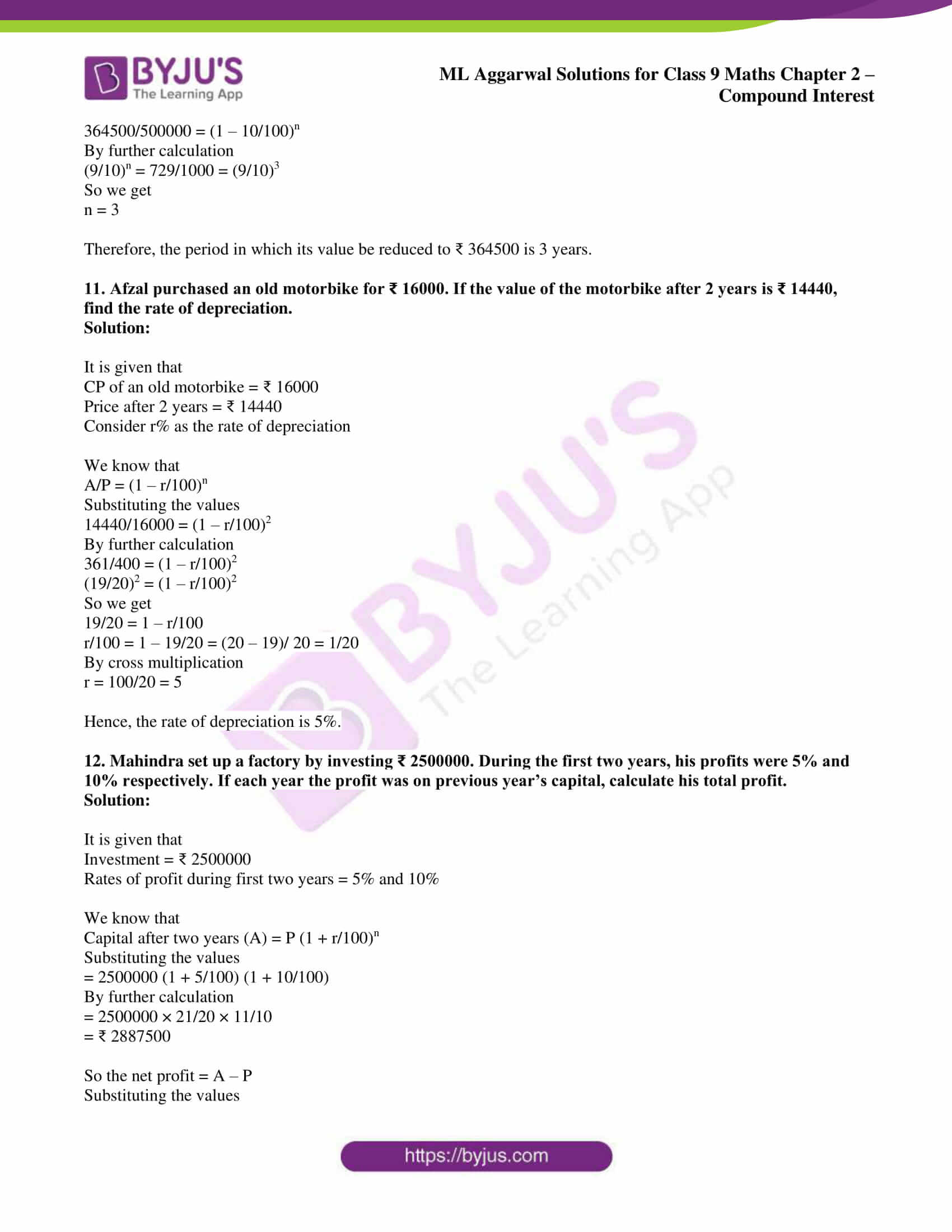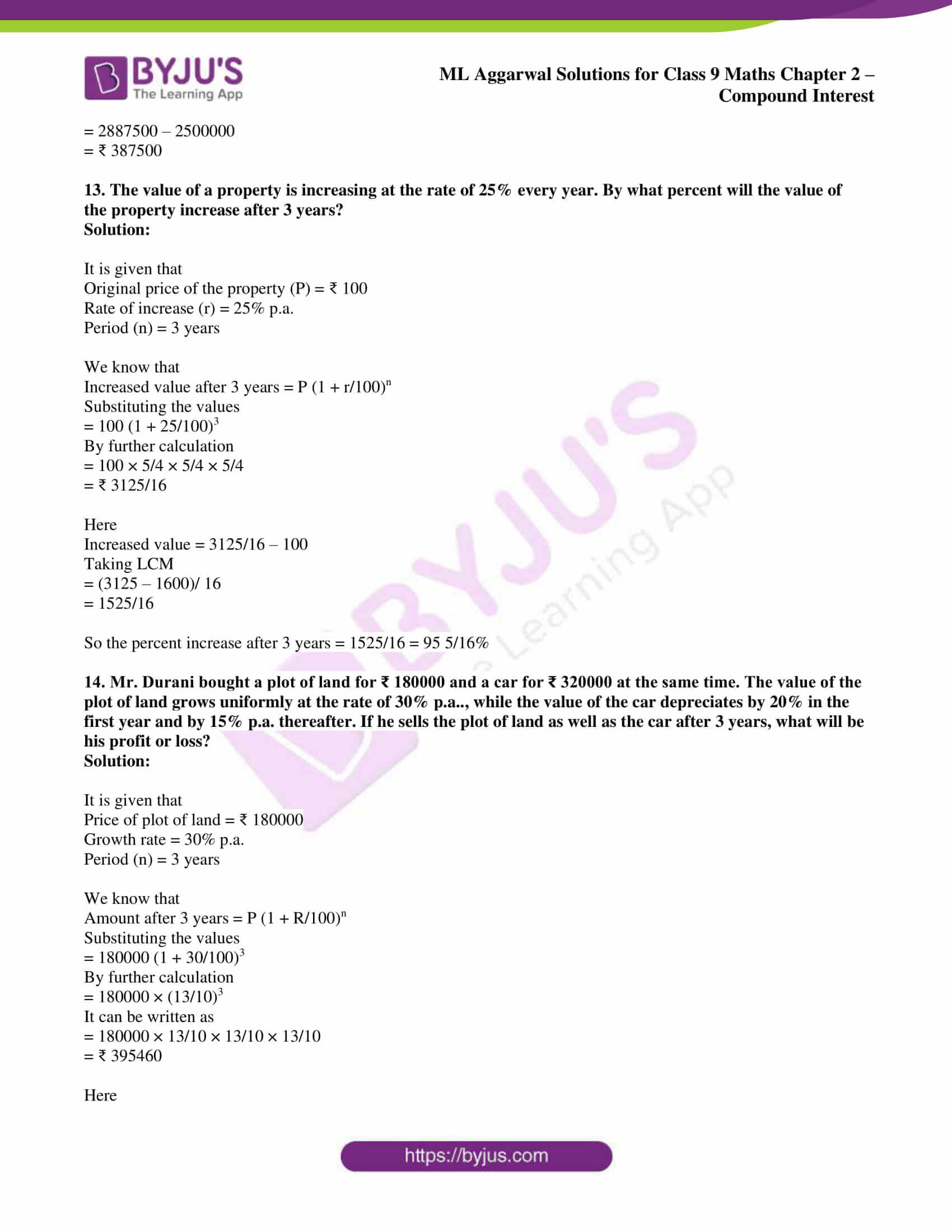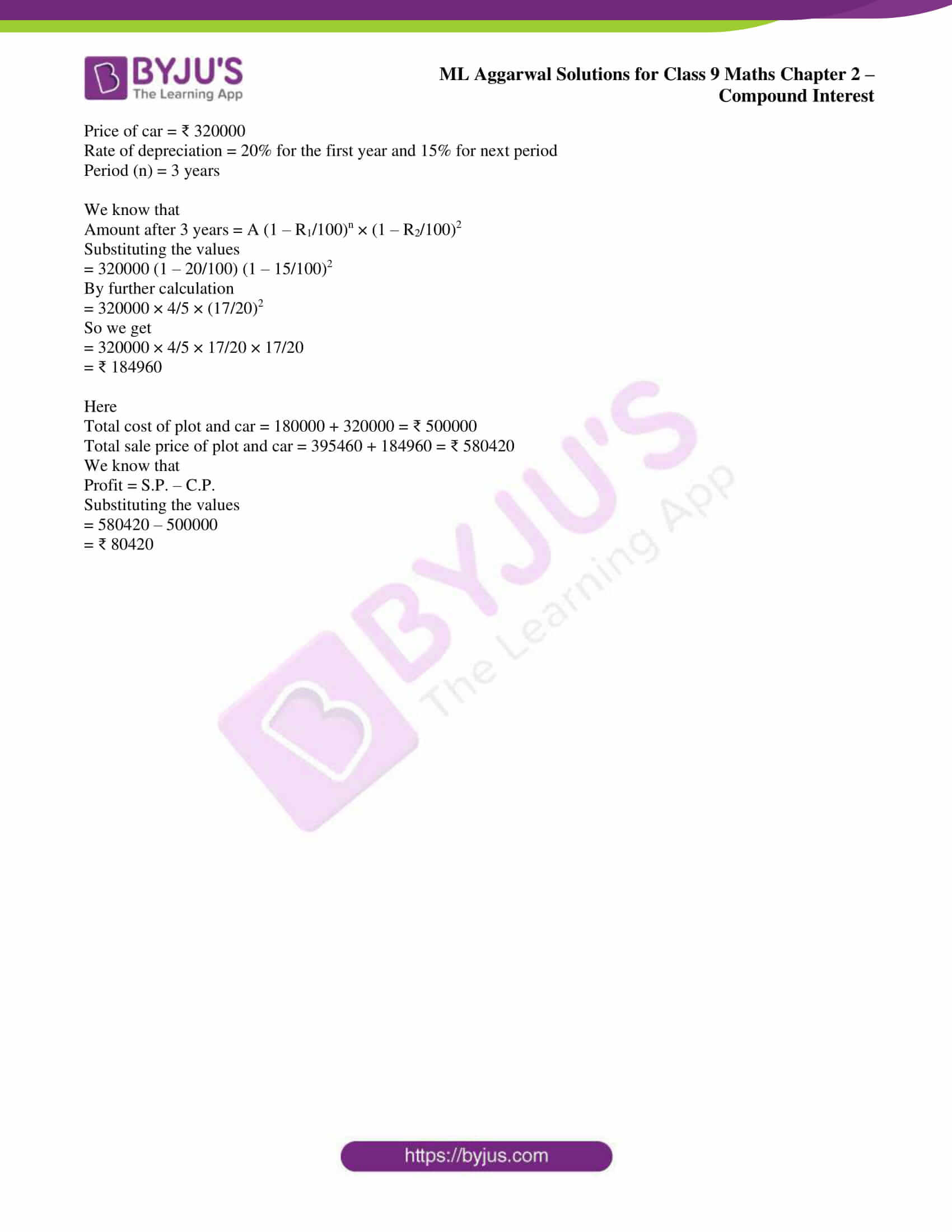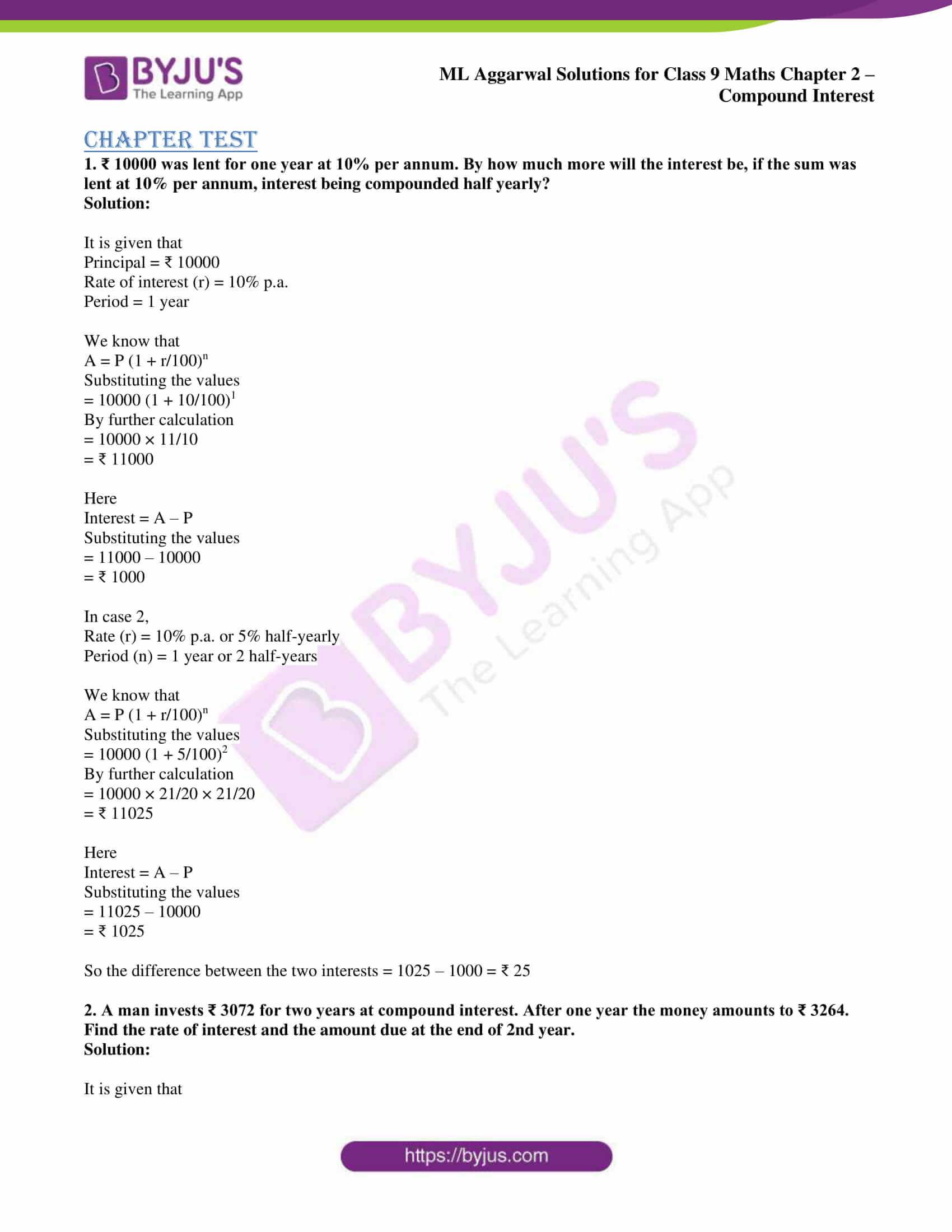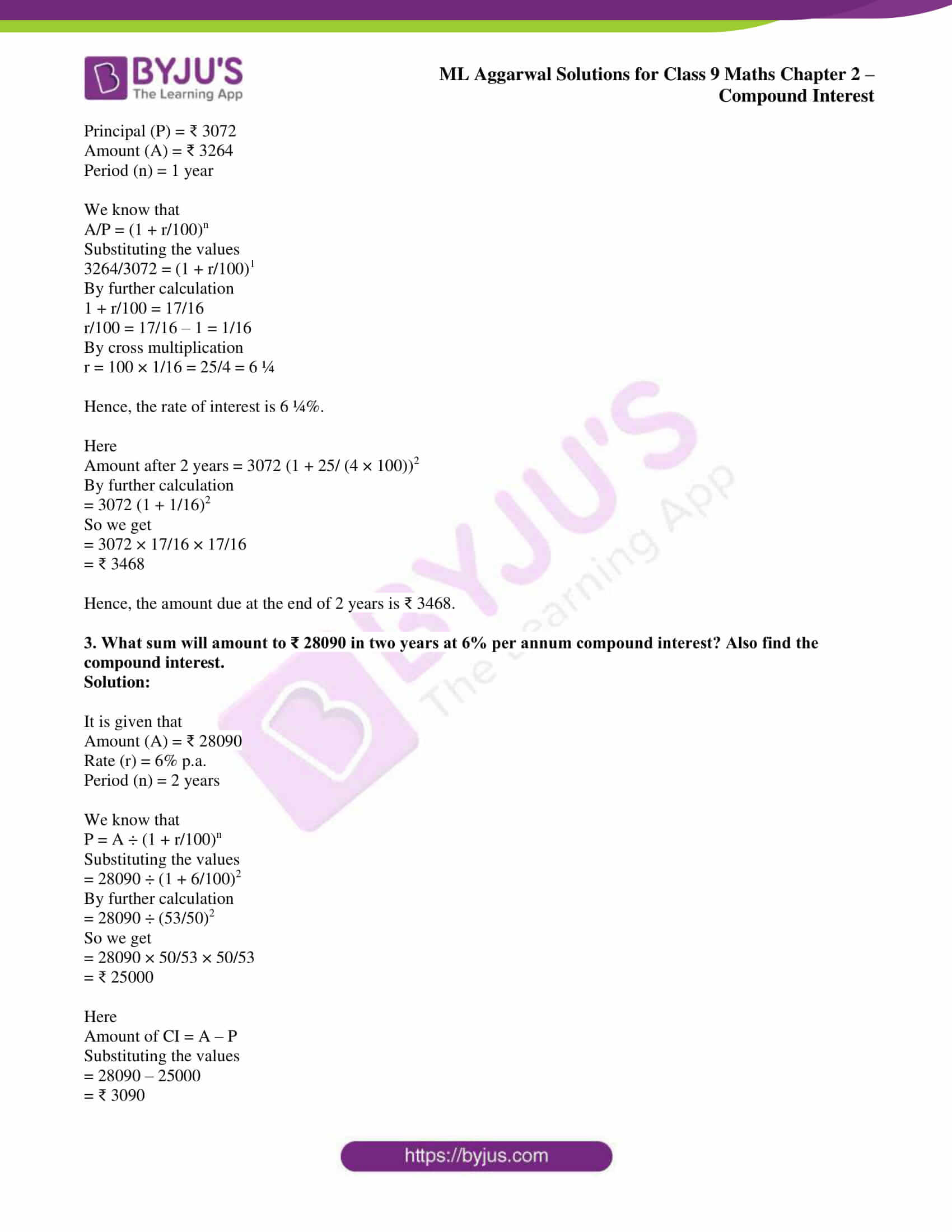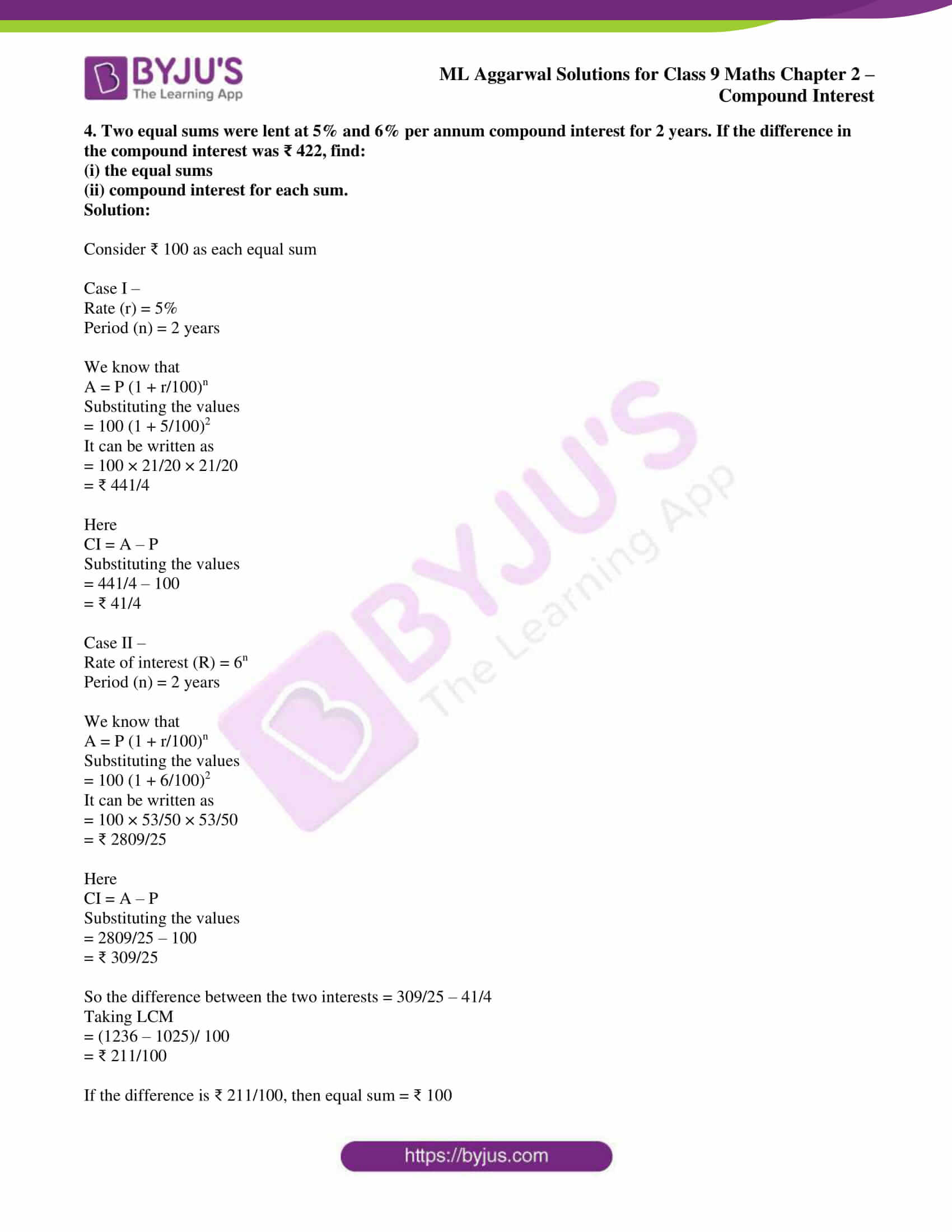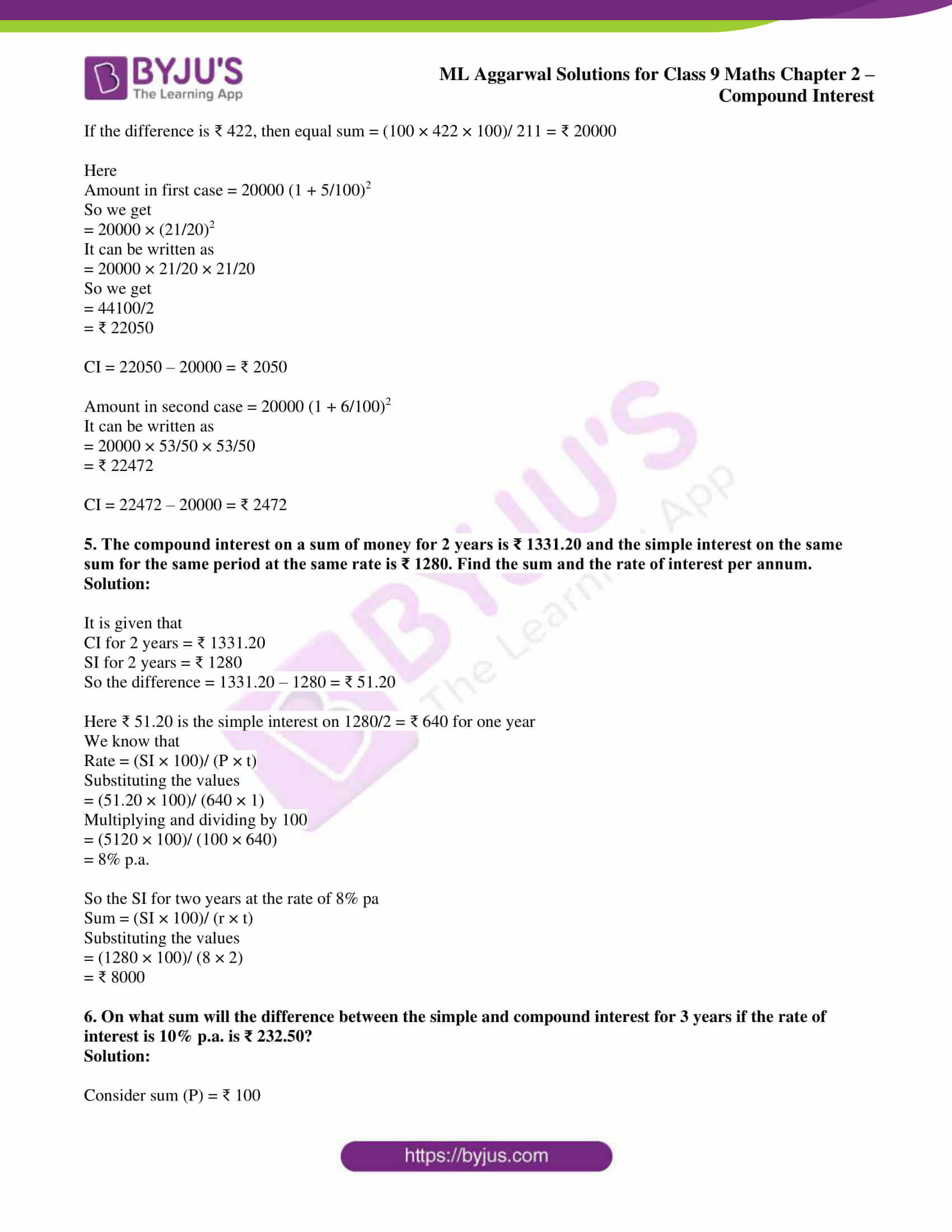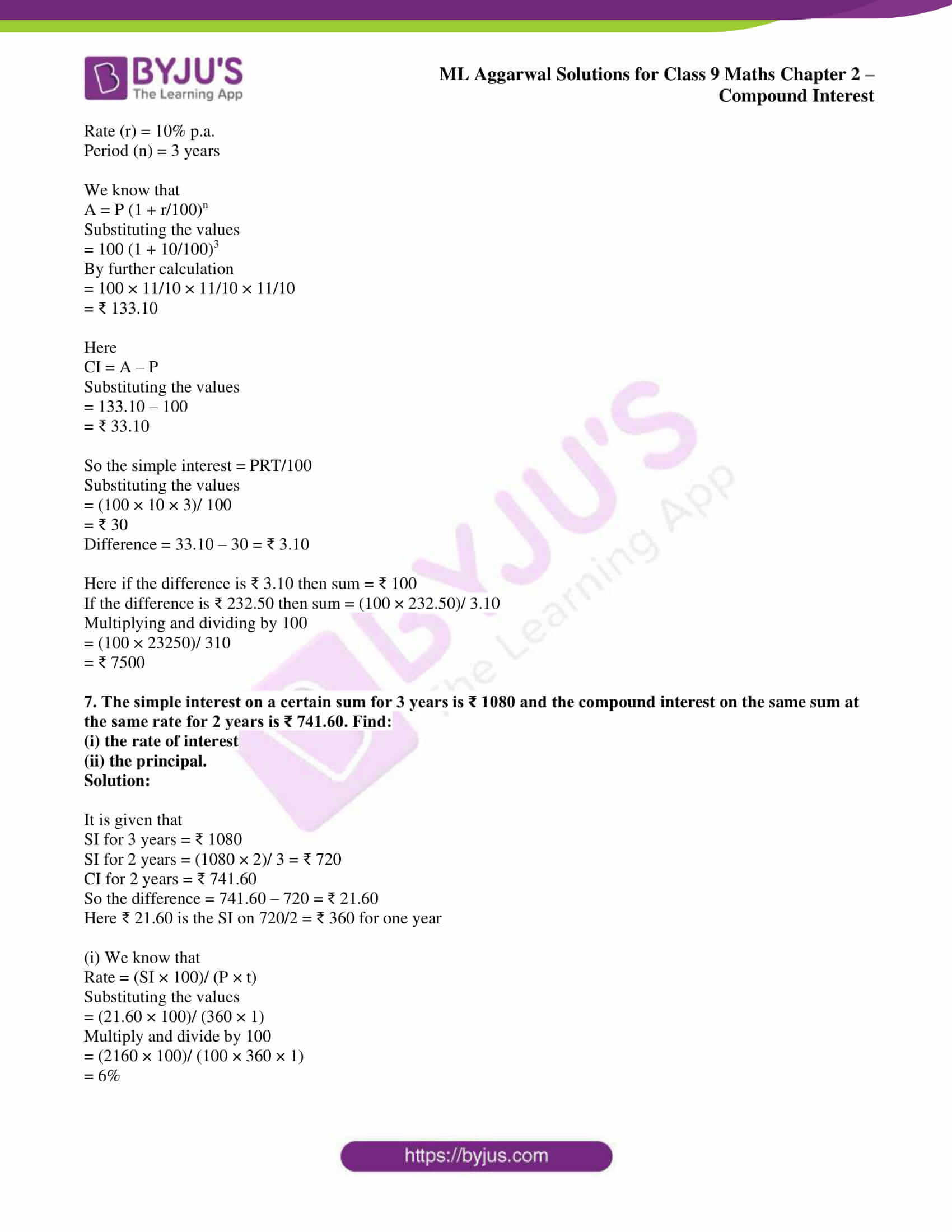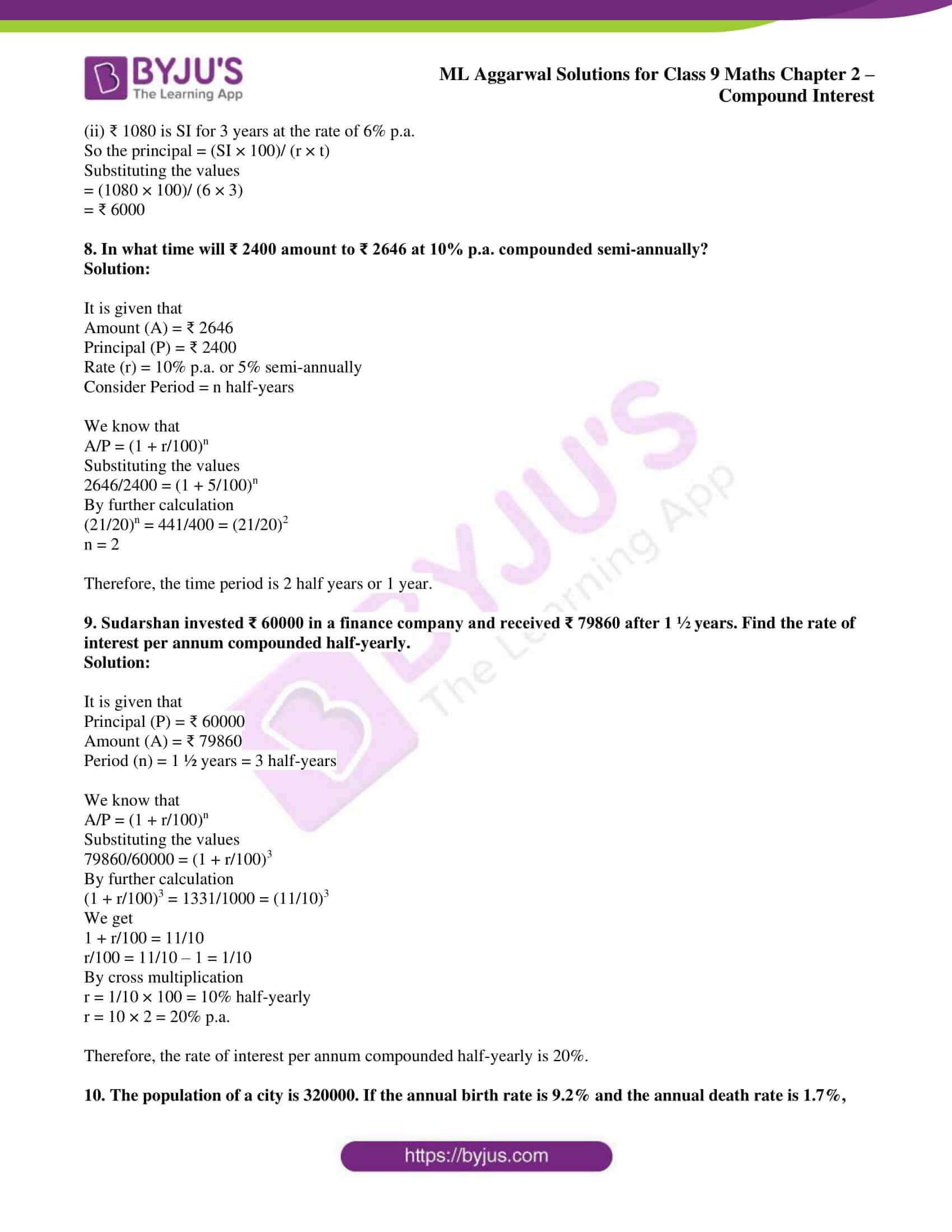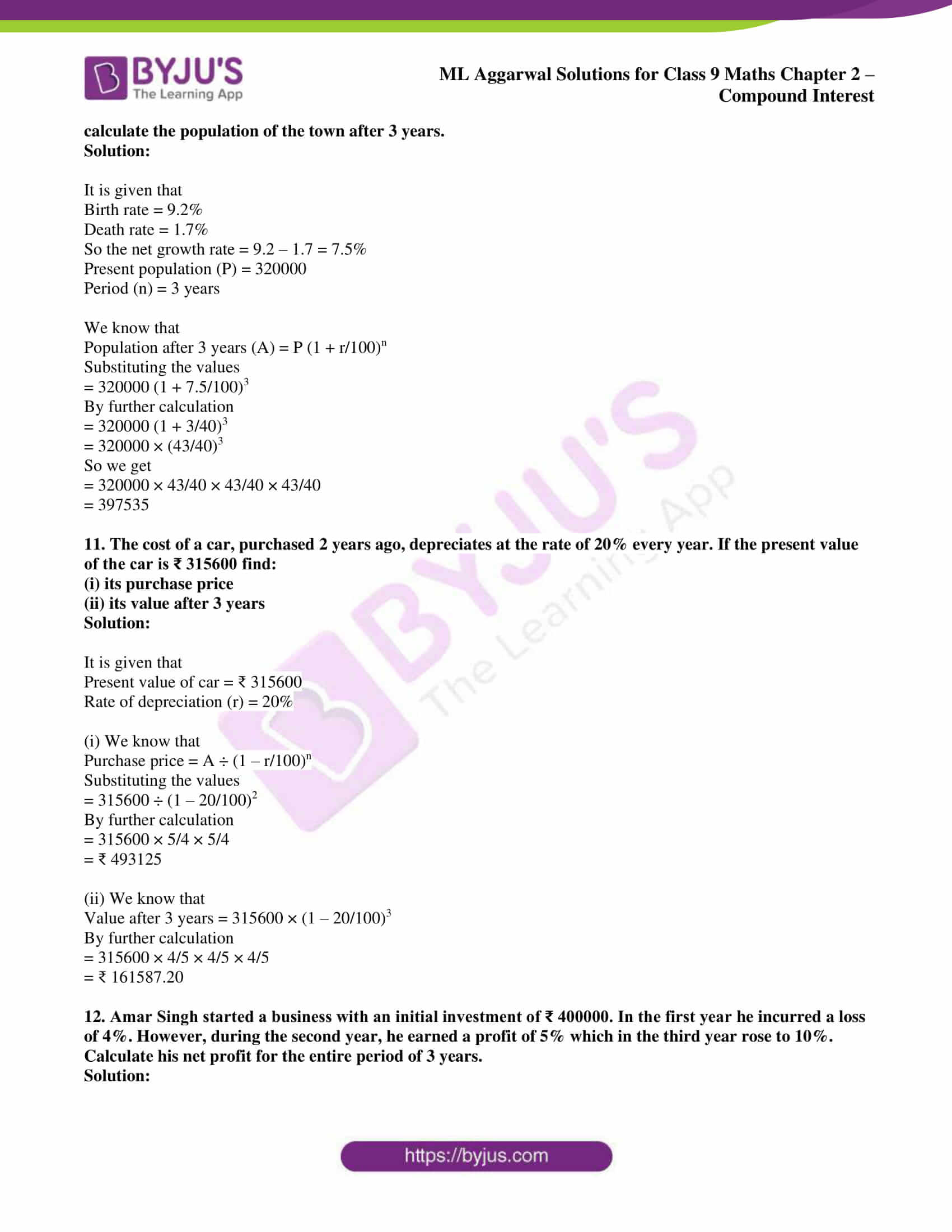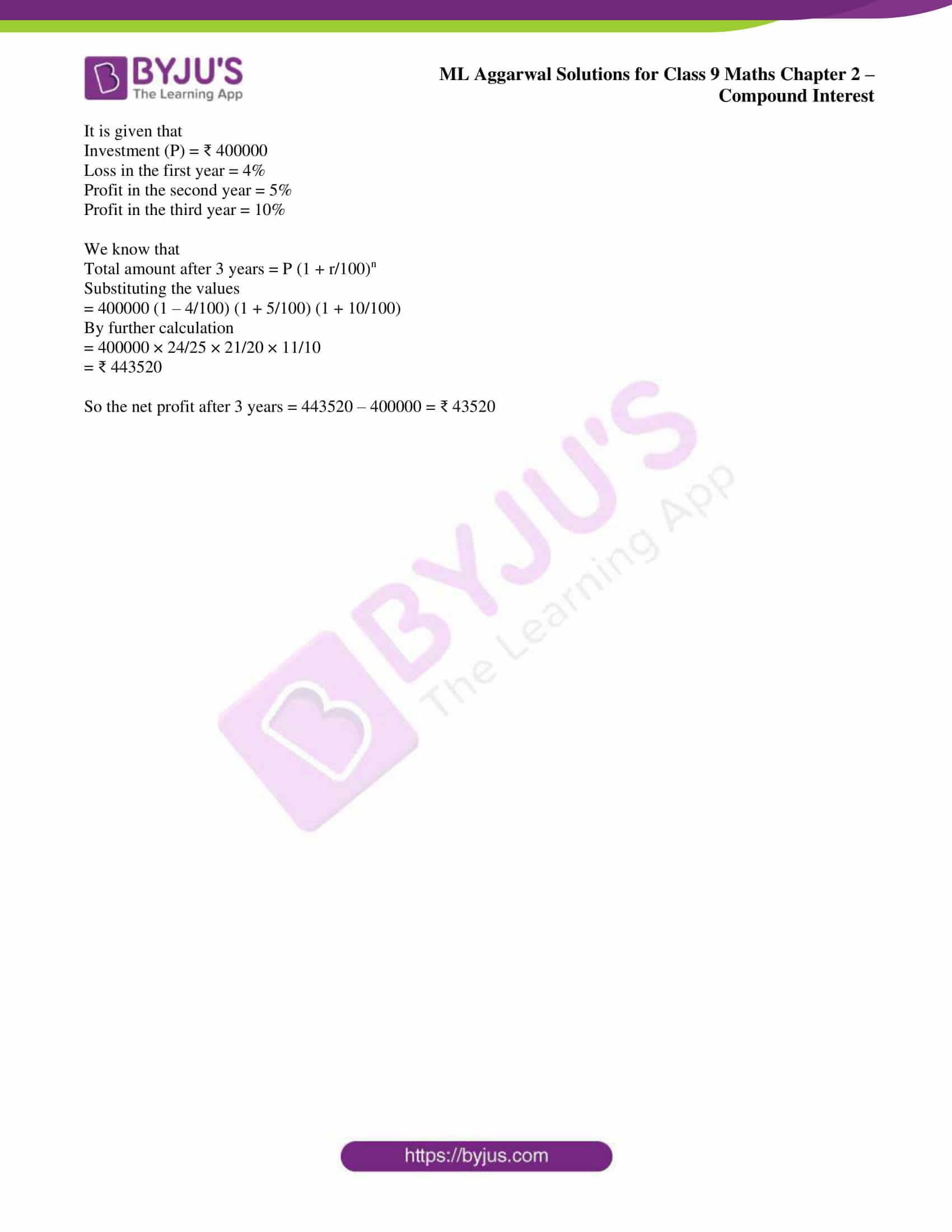## Access ML Aggarwal Solutions for Class 9 Maths Chapter 2: Compound Interest

Exercise 2.1

1. Find the amount and the compound interest on ₹ 8000 at 5% per annum for 2 years.

Solution:

It is given that

Principal = ₹ 8000

Rate of interest = 5% p.a.

We know that

Interest for the first year = Prt/100

Substituting the values

= (8000 × 5 × 1)/ 100

= ₹ 400

So the amount for the first year or principal for the second year = 8000 + 400 = ₹ 8400

Here

Interest for the second year = (8400 × 5 × 1)/ 100

So we get

= ₹ 420

We know that

Amount after the second year = 8400 + 420

= ₹ 8820

Total compound interest = 8820 + 8000

= ₹ 820

2. A man invests ₹ 46875 at 4% per annum compound interest for 3 years. Calculate:

(i) the amount standing to his credit at the end of the second year.

(ii) the interest for the third year.

(iii) the interest for the first year.

Solution:

It is given that

Principal = ₹ 46875

Rate of interest = 4% p.a.

(i) Interest for the first year = Prt/100

Substituting the values

= (46875 × 4 × 1)/ 100

= ₹ 1875

So the amount after first year or principal for the second year = 46875 + 1875 = ₹ 48750

Here

Interest for the second year = (48750 × 4 × 1)/ 100

So we get

= ₹ 1950

(ii) We know that

Amount at the end of second year = 48750 + 1950

= ₹ 50700

(iii) Interest for the third year = (50700 × 4 × 1)/ 100 = ₹ 2028

3. Calculate the compound interest for the second year on ₹ 8000 for three years at 10% p.a.

Also find the sum due at the end of third year.

Solution:

It is given that

Principal = ₹ 8000

Rate of interest = 10% p.a.

We know that

Interest for the first year = Prt/100

Substituting the values

= (8000 × 10 × 1)/ 100

= ₹ 800

So the amount after the first year or principal for the second year = 8000 + 800 = ₹ 8800

(i) Interest for the second year = (8800 × 10 × 1)/ 100

= ₹ 880

So the amount after second year or principal for the third year = 8800 + 880 = ₹ 9680

Interest for the third year = (9680 × 10 × 1)/ 100

= ₹ 968

(ii) Amount due at the end of the third year = 9680 + 968

= ₹ 10648

4. Ramesh invests ₹ 12800 for three years at the rate of 10% per annum compound interest.

Find:

(i) the sum due to Ramesh at the end of the first year.

(ii) the interest he earns for the second year.

(iii) the total amount due to him at the end of three years.

Solution:

It is given that

Principal = ₹ 12800

Rate of interest = 10% p.a.

(i) We know that

Interest for the first year = (12800 × 10 × 1)/ 100

= ₹ 1280

So the sum due at the end of first year = 12800 + 1280

= ₹ 14080

(ii) Principal for second year = ₹ 14080

So the interest for the second year = (14080 × 10 × 1)/ 100

= ₹ 1408

(iii) We know that

Sum due at the end of second year = 14080 + 1408

= ₹ 15488

Here

Principal for third year = ₹ 15488

Interest for the third year = (15488 × 10 × 1)/ 100

= ₹ 1548.80

So the total amount due to him at the end of third year = 15488 + 1548.80

= ₹ 17036.80

5. The simple interest on a sum of money for 2 years at 12% per annum is ₹ 1380. Find:

(i) the sum of money.

(ii) the compound interest on this sum for one year payable half-yearly at the same rate.

Solution:

It is given that

Simple Interest (SI) = ₹ 1380

Rate of interest (R) = 12% p.a.

Period (T) = 2 years

(i) We know that

Sum (P) = (SI × 100)/ (R × T)

Substituting the values

= (1380 × 100)/ (12 × 2)

= ₹ 5750

(ii) Here

Principal (P) = ₹ 5750

Rate of interest (R) = 12% p.a. or 6% half-yearly

Period (n) = 1 year – 2 half years

So we get

Amount (A) = P (1 + R/100)n

Substituting the values

= 5750 (1 + 6/100)2

By further calculation

= 5750 × (53/50)2

So we get

= 5750 × 53/50 × 53/50

= ₹ 6460.70

Here

Compound Interest = A – P

Substituting the values

= 6460.70 – 5750

= ₹ 710.70

6. A person invests ₹ 10000 for two years at a certain rate of interest, compounded annually. At the end of one year this sum amounts to ₹ 11200. Calculate:

(i) the rate of interest per annum.

(ii) the amount at the end of second year.

Solution:

It is given that

Principal (P) = ₹ 10,000

Period (T) = 1 year

Sum amount (A) = ₹ 11,200

Rate of interest = ?

(i) We know that

Interest (I) = 11200 – 10000 = ₹ 1200

So the rate of interest

R = (I × 100)/ (P × T)

Substituting the values

R = (1200 × 100)/ (10000 × 1)

So we get

R = 12% p.a.

Therefore, the rate of interest per annum is 12% p.a.

(ii) We know that

Period (T) = 2 years

Rate of interest (R) = 12% p.a.

Here

A = P (1 + R/100)t

Substituting the values

A = 10000 (1 + 12/100)2

By further calculation

A = 10000 (28/25)2

We can write it as

A = 10000 × 28/25 × 28/25

So we get

A = 16 × 28 × 28

A = ₹ 12544

Therefore, the amount at the end of second year is ₹ 12544.

7. Mr. Lalit invested ₹ 75000 at a certain rate of interest, compounded annually for two years. At the end of first year it amounts to ₹ 5325. Calculate

(i) the rate of interest.

(ii) the amount at the end of second year, to the nearest rupee.

Solution:

It is given that

Investment of Mr. Lalit = ₹ 5000

Period (n) = 2 years

(i) We know that

Amount after one year = ₹ 5325

So the interest for the first year = A – P

Substituting the values

= 5325 – 5000

= ₹ 325

Here

Rate = (SI × 100)/ (P × T)

Substituting the values

= (325 × 100)/ (5000 × 1)

So we get

= 13/2

= 6.5 % p.a.

(ii) We know that

Interest for the second year = (5325 × 13 × 1)/ (100 × 2)

By further calculation

= (213 × 13)/ (4 × 2)

So we get

= 2769/8

= ₹ 346.12

So the amount after second year = 5325 + 346.12

We get

= ₹ 5671.12

= ₹ 5671 (to the nearest rupee)

8. A man invests ₹ 5000 for three years at a certain rate of interest, compounded annually. At the end of one year it amounts to ₹ 5600. Calculate:

(i) the rate of interest per annum

(ii) the interest accrued in the second year.

(iii) the amount at the end of the third year.

Solution:

It is given that

Principal = ₹ 5000

Consider r% p.a. as the rate of interest

(i) We know that

At the end of one year

Interest = Prt/100

Substituting the values

= (5000 × r × 1)/ 100

= 50r

Here

Amount = 5000 + 50r

We can write it as

5000 + 50r = 5600

By further calculation

50r = 5600 – 5000 = 600

So we get

r = 600/50 = 12

Hence, the rate of interest is 12% p.a.

(ii) We know that

Interest for the second year = (5600 × 12 × 1)/ 100

= ₹ 672

So the amount at the end of second year = 5600 + 672

= ₹ 6272

(iii) We know that

Interest for the third year = (6272 × 12 × 1)/ 100

= ₹ 752.64

So the amount after third year = 6272 + 752.64

= ₹ 7024.64

9. Find the amount and the compound interest on ₹ 2000 at 10% p.a. for 2 years, compounded annually.

Solution:

It is given that

Principal (P) = ₹ 2000

Rate of interest (r) = 10% p.a.

Period (n) = 2 ½ years

We know that

Amount = P (1 + r/100)n

Substituting the values

= 2000 (1 + 10/100)2 (1 + 10/(2 × 100))

By further calculation

= 2000 × 11/10 × 11/10 × 21/20

So we get

= ₹ 2541

Here

Interest = A – P

Substituting the values

= 2541 – 2000

= ₹ 541

10. Find the amount and the compound interest on ₹ 50000 for 1 ½ years at 8% per annum, the interest being compounded semi-annually.

Solution:

It is given that

Principal (P) = ₹ 50000

Rate of interest (r) = 8% p.a. = 4% semi-annually

Period (n) = 1 ½ years = 3 semi-annually

We know that

Amount = P (1 + r/100)n

Substituting the values

= 50000 (1 + 4/100)3

By further calculation

= 50000 (26/25)3

= 50000 × 26/25 × 26/25 × 26/25

= ₹ 56243.20

Here

Compound Interest = A – P

Substituting the values

= 56243.20 – 50000

= ₹ 6243.20

11. Calculate the amount and the compound interest on ₹ 5000 in 2 years when the rate of interest for successive years is 6% and 8%, respectively.

Solution:

It is given that

Principal = ₹ 5000

Period = 2 years

Rate of interest for the first year = 6%

Rate of interest for the second year = 8%

We know that

Amount for two years = P (1 + r/100)n

Substituting the values

= 5000 (1 + 6/100) (1 + 8/100)

By further calculation

= 5000 × 53/50 × 27/25

= ₹ 5724

Here

Interest = A – P

Substituting the values

= 5724 – 5000

= ₹ 724

12. Calculate the amount and the compound interest on ₹ 17000 in 3 years when the rate of interest for successive years is 10%, 10% and 14%, respectively.

Solution:

It is given that

Principal = ₹ 17000

Period = 3 years

Rate of interest for 3 successive years = 10%, 10% and 14%

We know that

Amount after 3 years = P (1 + r/100)n

Substituting the values

= 17000 (1 + 10/100) (1 + 10/100) (1 + 14/100)

By further calculation

= 17000 × 11/10 × 11/10 × 57/50

= ₹ 23449.80

Here

Amount of compound interest = A – P

Substituting the values

= 23449.80 – 17000

= ₹ 6449.80

13. A sum of ₹ 9600 is invested for 3 years at 10% per annum at compound interest.

(i) What is the sum due at the end of the first year?

(ii) What is the sum due at the end of the second year?

(iii) Find the compound interest earned in 2 years.

(iv) Find the difference between the answers in (ii) and (i) and find the interest on this sum for one year.

(v) Hence, write down the compound interest for the third year.

Solution:

It is given that

Principal = ₹ 9600

Rate of interest = 10% p.a.

Period = 3 years

We know that

Interest for the first year = Prt/100

Substituting the values

= (9600 × 10 × 1)/ 100

= ₹ 960

(i) Amount after one year = 9600 – 960 = ₹ 10560

So the principal for the second year = ₹ 10560

Here the interest for the second year = (10560 × 10 × 1)/ 100

= ₹ 1056

(ii) Amount after two years = 10560 + 1056 = ₹ 11616

(iii) Compound interest earned in 2 years = 960 + 10560 = ₹ 2016

(iv) Difference between the answers in (ii) and (i) = 11616 – 10560 = ₹ 1056

We know that

Interest on ₹ 1056 for 1 year at the rate of 10% p.a. = (1056 × 10 × 1)/ 100

= ₹ 105.60

(v) Here

Principal for the third year = ₹ 11616

So the interest for the third year = (11616 × 10 × 1)/ 100

= ₹ 1161.60

14. The simple interest on a certain sum of money for 2 years at 10% p.a. is ₹ 1600. Find the amount due and the compound interest on this sum of money at the same rate after 3 years, interest being reckoned annually.

Solution:

It is given that

Period = 2 years

Rate = 10% p.a.

We know that

Sum = (SI × 100)/ (r × n)

Substituting the values

= (1600 × 100)/ (10 × 2)

= ₹ 8000

Here

Amount after 3 years = P (1 + r/100)n

Substituting the values

= 8000 (1 + 10/100)3

By further calculation

= 8000 × 11/10 × 11/10 × 11/10

= ₹ 10648

So the compound interest = A – P

Substituting the values

= 10648 – 8000

= ₹ 2648

15. Vikram borrowed ₹ 20000 from a bank at 10% per annum simple interest. He lent it to his friend Venkat at the same rate but compounded annually. Find his gain after 2 ½ years.

Solution:

First case-

Principal = ₹ 20000

Rate = 10% p.a.

Period = 2 ½ = 5/2 years

We know that

Simple interest = Prt/100

Substituting the values

= (20000 × 10 × 5)/ (100 × 2)

= ₹ 5000

Second case-

Principal = ₹ 20000

Rate = 10% p.a.

Period = 2 ½ years at compound interest

We know that

Amount = P (1 + r/100)n

Substituting the values

= 20000 (1 + 10/100)2 (1 + 10/ (2 × 100))2

By further calculation

= 20000 × 11/10 × 11/10 × 21/20

= ₹ 25410

Here

Compound Interest = A – P

Substituting the values

= 25410 – 20000

= ₹ 5410

So his gain after 2 years = CI – SI

We get

= 5410 – 5000

= ₹ 410

16. A man borrows ₹ 6000 at 5% compound interest. If he repays ₹ 1200 at the end of each year, find the amount outstanding at the beginning of the third year.

Solution:

It is given that

Principal = ₹ 6000

Rate of interest = 5% p.a.

We know that

Interest for the first year = Prt/100

Substituting the values

= (6000 × 5 × 1)/ 100

= ₹ 300

So the amount after one year = 6000 + 300 = ₹ 6300

Principal for the second year = ₹ 6300

Amount paid = ₹ 1200

So the balance = 6300 – 1200 = ₹ 5100

Here

Interest for the second year = (5100 × 5 × 1)/ 100 = ₹ 255

Amount for the second year = 5100 + 255 = ₹ 5355

Amount paid = ₹ 1200

So the balance = 5355 – 1200 = ₹ 4155

17. Mr. Dubey borrows ₹ 100000 from State Bank of India at 11% per annum compound interest. He repays ₹ 41000 at the end of first year and ₹ 47700 at the end of second year. Find the amount outstanding at the beginning of the third year.

Solution:

It is given that

Borrowed money (P) = ₹ 100000

Rate = 11% p.a.

Time = 1 year

We know that

Amount after first year = Prt/100

Substituting the values

= (100000 × 11 × 1)/ 100

By further calculation

= 100000 + 11000

= ₹ 111000

Amount paid at the end of first year = ₹ 41000

So the principal for second year = 111000 – 41000

= ₹ 70000

We know that

Amount after second year = P + (70000 × 11)/ 100

By further calculation

= 70000 + 700

= ₹ 77700

So the amount paid at the end of second year = ₹ 47700

Here the amount outstanding at the beginning year = 77700 – 47700

= ₹ 30000

18. Jaya borrowed ₹ 50000 for 2 years. The rates of interest for two successive years are 12% and 15% respectively. She repays ₹ 33000 at the end of first year. Find the amount she must pay at the end of second year to clear her debt.

Solution:

It is given that

Amount borrowed by Jaya = ₹ 50000

Period (n) = 2 years

Rate of interest for two successive years are 12% and 15% respectively

We know that

Interest for the first year = Prt/100

Substituting the values

= (50000 × 12 × 1)/ 100

= ₹ 6000

So the amount after first year = 50000 + 6000 = ₹ 56000

Amount repaid = ₹ 33000

Here

Balance amount for the second year = 56000 – 33000 = ₹ 23000

Rate = 15%

So the interest for the second year = (230000 × 15 × 1)/ 100

= ₹ 3450

Amount paid after second year = 23000 + 3450 = ₹ 26450

Exercise 2.2

1. Find the amount and the compound interest on ₹ 5000 for 2 years at 6% per annum, interest payable yearly.

Solution:

It is given that

Principal (P) = ₹ 5000

Rate of interest (r) = 6% p.a.

Period (n) = 2 years

We know that

Amount = P (1 + r/100)n

Substituting the values

= 5000 (1 + 6/100)2

By further calculation

= 5000 × 53/50 × 53/50

= ₹ 5618

Here

CI = A – P

Substituting the values

= 5618 – 5000

= ₹ 618

2. Find the amount and the compound interest on ₹ 8000 for 4 years at 10% per annum interest reckoned yearly.

Solution:

It is given that

Principal (P) = ₹ 8000

Rate of interest (r) = 10% p.a.

Period (n) = 4 years

We know that

Amount = P (1 + r/100)n

Substituting the values

= 8000 (1 + 10/100)4

By further calculation

= 8000 × 11/10 × 11/10 × 11/10 × 11/10

= ₹ 11712.80

Here

CI = A – P

Substituting the values

= 11712.80 – 8000

= ₹ 3712.80

3. If the interest is compounded half yearly, calculate the amount when the principal is ₹ 7400, the rate of interest is 5% and the duration is one year.

Solution:

It is given that

Principal (P) = ₹ 7400

Rate of interest (r) = 5%

Period (n) = 1 year

We know that

A = P (1 + r/(2 × 100))2×n

Substituting the values

= 7400 (1 + 5/200)2

By further calculation

= 7400 × 205/200 × 205/200

= ₹ 7774.63

4. Find the amount and the compound interest on ₹ 5000 at 10% p.a. for 1 ½ years, compound interest reckoned semi-annually.

Solution:

It is given that

Principal (P) = ₹ 5000

Rate of interest = 10% p.a. or 5% half-yearly

Period (n) = 1 ½ years or 3 half-years

We know that

A = P (1 + r/100)n

Substituting the values

= 5000 (1 + 5/100)3

By further calculation

= 5000 × 21/20 × 21/20 × 21/20

= ₹ 5788.12

Here

CI = A – P

Substituting the values

= 5788.12 – 5000

= ₹ 788.12

5. Find the amount and the compound interest on ₹ 100000 compounded quarterly for 9 months at the rate of 4% p.a.

Solution:

It is given that

Principal (P) = ₹ 100000

Rate of interest = 4% p.a. or 1% quarterly

Period (n) = 9 months or 3 quarters

We know that

A = P (1 + r/100)n

Substituting the values

= 100000 (1 + 1/100)3

By further calculation

= 100000 × 101/100 × 101/100 × 101/100

= ₹ 103030.10

Here

CI = A – P

Substituting the values

= 103030.10 – 100000

= ₹ 3030.10

6. Find the difference between CI and SI on sum of ₹ 4800 for 2 years at 5% per annum payable yearly.

Solution:

It is given that

Principal (P) = ₹ 4800

Rate of interest (r) = 5% p.a.

Period (n) = 2 years

We know that

SI = Prt/100

Substituting the values

= (4800 × 5 × 2)/ 100

= ₹ 480

If compounded yearly

A = P (1 + r/100)n

Substituting the values

= 4800 (1 + 5/100)2

By further calculation

= 4800 × 21/20 × 21/20

= ₹ 5292

Here

CI = A – P

Substituting the values

= 5292 – 4800

= ₹ 492

So the difference between CI and SI = 492 – 480 = ₹ 12

7. Find the difference between the simple interest and compound interest on ₹ 2500 for 2 years at 4% per annum, compound interest being reckoned semi-annually.

Solution:

It is given that

Principal (P) = ₹ 2500

Rate of interest (r) = 4% p.a. or 2% half-yearly

Period (n) = 2 years or 4 half-years

We know that

SI = Prt/100

Substituting the values

= (2500 × 4 × 2)/100

= ₹ 200

If compounded semi-annually

A = P (1 + r/100)n

Substituting the values

= 2500 (1 + 2/100)4

By further calculation

= 2500 × 51/50 × 51/50 × 51/50 × 51/50

= ₹ 2706.08

We know that

CI = A – P

Substituting the values

= 2706.08 – 2500

= ₹ 206.08

So the difference between CI and SI = 206.08 – 200 = ₹ 6.08

8. Find the amount and the compound interest on ₹ 2000 in 2 years if the rate is 4% for the first year and 3% for the second year.

Solution:

It is given that

Principal (P) = ₹ 2000

Rate of interest = 4% on the first year and 3% for the second year

Period (n) = 2 years

We know that

Amount = P (1 + r/100)n

Substituting the values

= 2000 (1 + 4/100) (1 + 3/100)

By further calculation

= 2000 × 26/25 × 103/100

= ₹ 2142.40

Here

CI = A – P

Substituting the values

= 2142.40 – 2000

= ₹ 142.40

9. Find the compound interest on ₹ 3125 for 3 years if the rates of interest for the first, second and third year are respectively 4%, 5% and 6% per annum.

Solution:

It is given that

Principal (P) = ₹ 3125

Rate of interest for continuous = 4%, 5% and 6%

Period (n) = 3 years

We know that

Amount = P (1 + r/100)n

Substituting the values

= 3125 (1 + 4/100) (1 + 5/100) (1 + 6/100)

By further calculation

= 3125 × 26/25 × 21/50 × 53/50

= ₹ 3617.25

Here

CI = A – P

Substituting the values

= 3617.25 – 3125

= ₹ 492.25

10. What sum of money will amount to ₹ 9261 in 3 years at 5% per annum compound interest?

Solution:

It is given that

Amount (A) = ₹ 9261

Rate of interest (r) = 5% per annum

Period (n) = 3 years

We know that

A = P (1 + r/100)n

Substituting the values

9261 = P (1 + 5/100)3

By further calculation

9261 = P (21/20)3

So we get

P = (9261 × 20 × 20 × 20)/ (21 × 21 × 21)

P = ₹ 8000

Therefore, the sum of money is ₹ 8000.

11. What sum invested at 4% per annum compounded semi-annually amounts to ₹ 7803 at the end of one year?

Solution:

It is given that

Amount (A) = ₹ 7803

Rate of interest (r) = 4% p.a. or 2% semi-annually

Period (n) = 1 year or 2 half years

We know that

A = P (1 + r/100)n

Substituting the values

= 7803 + (1 + 2/100)2

By further calculation

= 7803 + (51/20)2

= 7803 × 50/51 × 50/51

= ₹ 7500

Hence, the principal is ₹ 7500.

12. What sum invested for 1 ½ years compounded half yearly at the rate of 4% p.a. will amount to ₹132651?

Solution:

It is given that

Amount (A) = ₹ 132651

Rate of interest (r) = 4% p.a. or 2% half yearly

Period (n) = 1 ½ years or 3 half years

We know that

A = P (1 + r/100)n

It can be written as

P = A ÷ (1 + r/100)n

Substituting the values

= 132651 ÷ (1 + 2/100)3

By further calculation

= 132651 ÷ (51/50)3

So we get

= 132651 × (50/51)3

= 132651 × 50/51 × 50/51 × 50/51

= ₹ 125000

Hence, the principal amount is ₹ 125000.

13. On what sum will the compound interest for 2 years at 4% per annum be ₹ 5712?

Solution:

It is given that

CI = ₹ 5712

Rate of interest (r) = 4% p.a.

Period (n) = 2 years

We know that

A = P (1 + r/100)n

It can be written as

CI = A – P = P (1 + r/100)n – P

= P [(1 + r/100)n – 1]

Substituting the values

5712 = P [(1 + 4/100)2 – 1]

= P [(26/25)2 – 1]

By further calculation

= P [676/625 – 1]

Taking LCM

= P [(676 – 625)/ 625]

= P × 51/625

Here

P = 5712 × 625/51

= 112 × 625

= ₹ 70000

Hence, the principal amount is ₹ 70000.

14. A man invests ₹ 1200 for two years at compound interest. After one year the money amounts to ₹ 1275. Find the interest for the second year correct to the nearest rupee.

Solution:

It is given that

Principal = ₹ 1200

After one year, the amount = ₹ 1275

So the interest for one year = 1275 – 1200 = ₹ 75

We know that

Rate of interest = (SI × 100)/ (P × t)

Substituting the values

= (75 × 100)/ (1200 × 1)

By further calculation

= 75/12

= 25/4

= 6 ¼ % p.a.

Here

Interest for the second year on ₹ 1275 at the rate of 25/4% = Prt/100

Substituting the values

= (1275 × 25 × 1)/ (100 × 4)

By further calculation

= 1275/16

= ₹ 79.70

= ₹ 80

15. At what rate percent per annum compound interest will ₹ 2304 amount to ₹ 2500 in 2 years?

Solution:

It is given that

Amount = ₹ 2500

Principal = ₹ 2304

Period (n) = 2 years

Consider r% p.a. as the rate of interest

We know that

A = P (1 + r/100)n

It can be written as

(1 + r/100)n = A/P

Substituting the values

(1 + r/100)2 = 2500/2304

By further calculation

(1 + r/100)2 = 625/576 = (25/24)2

So we get

1 + r/100 = 25/24

r/100 = 25/24 – 1

Taking LCM

r = 100/24 = 25/6 = 4 1/6

Hence, the rate of interest is 4 1/6% p.a.

16. A sum compounded annually becomes 25/16 time of itself in two years. Determine the rate of interest per annum.

Solution:

Consider sum (P) = x

Amount (A) = 25/16x

Period (n) = 2 years

We know that

A/P = (1 + r/100)n

Substituting the values

25x/16x = (1 + r/100)2

By further calculation

(1 + r/100)2 = (5/4)2

So we get

1 + r/100 = 5/4

r/100 = 5/4 – 1/1 = 1/4

By cross multiplication

r = 100 × ¼ = 25

Hence, the rate of interest is 25% p.a.

17. At what rate percent will ₹ 2000 amount to ₹ 2315.25 in 3 years at compound interest?

Solution:

It is given that

Principal (P) = ₹ 2000

Amount (A) = ₹ 2315.25

Period (n) = 3 years

Consider r% p.a. as the rate of interest

We know that

A/P = (1 + r/100)n

Substituting the values

2315.25/2000 = (1 + r/100)3

By further calculation

(1 + r/100)3 = 231525/(100 × 2000) = 9261/8000 = (21/20)3

So we get

1 + r/100 = 21/20

It can be written as

r/100 = 21/20 – 1 = 1/20

r = 100/20 = 5

Hence, the rate of interest is 5% p.a.

18. If ₹ 40000 amounts to ₹ 48620.25 in 2 years, compound interest payable half-yearly, find the rate of interest per annum.

Solution:

It is given that

Principal (P) = ₹ 40000

Amount (A) = ₹ 48620.25

Period (n) = 2 years = 4 half years

Consider rate of interest = r% p.a. = r/2% half yearly

We know that

A/P = (1 + r/100)n

Substituting the values

48620.25/40000 = (1 + r/200)4

By further calculation

(1 + r/200)4 = 4862025/ (100 × 40000) = 194481/160000

So we get

(1 + r/200)4 = (21/20)4

It can be written as

1 + r/200 = 21/20

r/200 = 21/20 – 1 = 1/20

By cross multiplication

r = 200 × 1/20 = 10

Hence the rate of interest per annum is 10%.

19. Determine the rate of interest for a sum that becomes 216/125 times of itself in 1 ½ years, compounded semi-annually.

Solution:

Consider principal (P) = x

Amount (A) = 216/125 x

Period (n) = 1 ½ years = 3 half years

Take rate percent per year = 2r% and r% half yearly

We know that

A/P = (1 + r/100)n

Substituting the values

216x/125x = (1 + r/100)3

By further calculation

(1 + r/100)3 = 216/125 = (6/5)3

So we get

1 + r/100 = 6/5

r/100 = 6/5 – 1 = 1/5

By cross multiplication

r = 100 × 1/5 = 20%

So the rate percent per year = 2 × 20 = 40%

20. At what rate percent p.a. compound interest would ₹ 80000 amounts to ₹ 88200 in two years, interest being compounded yearly. Also find the amount after 3 years at the above rate of compound interest.

Solution:

It is given that

Principal (P) = ₹ 80000

Amount (A) = ₹ 88200

Period (n) = 2 years

Consider r% per annum as the rate of interest percent

We know that

A/P = (1 + r/100)n

Substituting the values

88200/80000 = (1 + r/100)2

By further calculation

(1 + r/100)2 = 441/400 = (21/20)2

So we get

1 + r/100 = 21/20

r/100 = 21/20 – 1 = 1/20

By cross multiplication

r = 1/20 × 100 = 5

Hence, the rate of interest is 5% per annum.

21. A certain sum amounts to ₹ 5292 in 2 years and to ₹ 5556.60 in 3 years at compound interest. Find the rate and the sum.

Solution:

It is given that

Amount after 2 years = ₹ 5292

Amount after 3 years = ₹ 5556.60

So the difference = 5556.60 – 5292 = ₹ 264.60

Here ₹ 264.60 is the interest on ₹ 5292 for one year

We know that

Rate % = (SI × 100)/ (P × t)

Substituting the values

= (264.60 × 100)/ (5292 × 1)

Multiply and divide by 100

= (26460 × 100)/ (100 × 5292)

= 5%

Here

A = P (1 + r/100)n

Substituting the values

5292 = P (1 + 5/100)2

By further calculation

P = 5292 ÷ (1 + 5/100)2

So we get

P = 5292 ÷ (21/20)2

P = 5292 × 21/20 × 21/20

P = ₹ 4800

Hence, the rate is 5% and the sum is ₹ 4800.

22. A certain sum amounts to ₹ 798.60 after 3 years and ₹ 878.46 after 4 years. Find the interest rate and the sum.

Solution:

It is given that

Amount after 3 years = ₹ 798.60

Amount after 4 years = ₹ 878.46

So the difference = 878.46 – 798.60 = ₹ 79.86

Here ₹ 79.86 is the interest on ₹ 798.60 for 1 year.

We know that

Rate = (SI × 100)/ (P × t)

Substituting the values

= (79.86 × 100)/ (798.60 × 1)

Multiply and divide by 100

= (7986 × 100 × 100)/ (79860 × 100 × 1)

= 10%

Here

A = P (1 + r/100)n

It can be written as

P = A ÷ (1 + r/100)n

Substituting the values

P = 798.60 ÷ (1 + 10/100)3

By further calculation

P = 79860/100 × 10/11 × 10/11 × 10/11

P = ₹ 600

23. In what time will ₹ 15625 amount to ₹ 17576 at 4% per annum compound interest?

Solution:

It is given that

Amount (A) = ₹ 17576

Principal (P) = ₹ 15625

Rate = 4% p.a.

Consider n years as the period

We know that

A/P = (1 + r/100)n

Substituting the values

17576/15625 = (1 + 4/100)n

By further calculation

(26/25)3 = (26/25)n

So we get

n = 3

24. (i) In what time will ₹ 1500 yield ₹ 496.50 as compound interest at 10% per annum compounded annually?

(ii) Find the time (in years) in which ₹ 12500 will produce ₹ 3246.40 as compound interest at 8% per annum, interest compounded annually.

Solution:

(i) It is given that

Principal (P) = ₹ 1500

CI = ₹ 496.50

So the amount (A) = P + SI

Substituting the values

= 1500 + 496.50

= ₹ 1996.50

Rate (r) = 10% p.a.

We know that

A = P (1 + r/100)n

It can be written as

A/P = (1 + r/100)n

Substituting the values

1996.50/1500 = (1 + 10/100)n

By further calculation

199650/(1500 × 100) = (11 /10)n

So we get

1331/1000 = (11/10)n

(11/10)3 = (11/10)n

Here Time n = 3 years

(ii) It is given that

Principal (P) = ₹ 12500

CI = ₹ 3246.40

So the amount (A) = P + CI

Substituting the values

= 12500 + 3246.40

= ₹ 15746.40

Rate (r) = 8% p.a.

We know that

A = P (1 + r/100)n

It can be written as

A/P = (1 + r/100)n

Substituting the values

15746.40/12500 = (1 + 8/100)n

Multiply and divide by 100

1574640/ (12500 × 100) = (27/25)n

By further calculation

78732/ (12500 × 5) = (27/ 25)n

19683/ (3125 × 5) = (27/25)n

So we get

19683/15625 = (27/25)n

(27/25)3 = (27/25)n

Here Period = 3 years

25. ₹ 16000 invested at 10% p.a., compounded semi-annually, amounts to ₹ 18522, find the time period of investment.

Solution:

It is given that

Principal (P) = ₹ 16000

Amount (A) = ₹ 18522

Rate = 10% p.a. or 5% semi-annually

Consider period = n half years

We know that

A/P = (1 + r/100)n

Substituting the values

18522/16000 = (1 + 5/100)n

By further calculation

9261/8000 = (21/20)n

So we get

(21/20)3 = (21/20)n

n = 3 half years

Here

Time = 3/2 = 1 ½ years

26. What sum will amount to ₹ 2782.50 in 2 years at compound interest, if the rates are 5% and 6% for the successive years?

Solution:

It is given that

Amount (A) = ₹ 2782.50

Rate of interest for two successive years = 5% and 6%

We know that

A = P (1 + r/100)n

Substituting the values

2782.50 = P (1 + 5/100) (1 + 6/100)

By further calculation

2782.50 = P × 21/20 × 53/50

So we get

P = 2782.50 × 20/21 × 50/53

Multiply and divide by 100

P = 278250/100 × 20/21 × 50/53

P = ₹ 2500

Hence, the principal is ₹ 2500.

27. A sum of money is invested at compound interest payable annually. The interest in two successive years is ₹ 225 and ₹ 240. Find:

(i) the rate of interest

(ii) the original sum

(iii) the interest earned in the third year.

Solution:

It is given that

Interest for the first year = ₹ 225

Interest for the second year = ₹ 240

So the difference = 240 – 225 = ₹ 15

Here ₹ 15 is the interest on ₹ 225 for 1 year

(i) Rate = (SI × 100)/ (P × t)

Substituting the values

= (15 × 100)/ (225 × 1)

So we get

= 20/3

= 6 2/3% p.a.

(ii) We know that

Sum = (SI × 100)/ (R × t)

Substituting the values

= (225 × 100)/ (20/3 × 1)

It can be written as

= (225 × 100 × 3)/ (20 × 1)

So we get

= 225 × 15

= ₹ 3375

(iii) Here

Amount after second year = 225 + 240 + 3375 = ₹ 3840

So the interest for the third year = Prt/100

Substituting the values

= (3840 × 20 × 1)/ (100 × 3)

= ₹ 256

28. On what sum of money will the difference between the compound interest and simple interest for 2 years be equal to ₹ 25 if the rate of interest charged for both is 5% p.a.?

Solution:

It is given that

Sum (P) = ₹ 100

Rate (R) = 5% p.a.

Period (n) = 2 years

We know that

SI = PRT/100

Substituting the values

= (100 × 5 × 2)/ 100

= ₹ 10

So the amount when interest is compounded annually = P (1 + R/100)n

Substituting the values

= 100 (1 + 5/100)2

By further calculation

= 100 × (21/20)2

= 100 × 21/20 × 21/20

So we get

= ₹ 441/4

Here

CI = A – P

Substituting the values

= 441/4 – 100

= ₹ 41/4

So the difference between CI and SI = 41/4 – 10 = ₹ ¼

If the difference is ₹ ¼ then sum = ₹ 100

If the difference is ₹ 25 then sum = (100 × 4)/ 1 × 25 = ₹ 10000

29. The difference between the compound interest for a year payable half-yearly and the simple interest on a certain sum of money lent out at 10% for a year is ₹ 15. Find the sum of money lent out.

Solution:

It is given that

Sum = ₹ 100

Rate = 10% p.a. or 5% half yearly

Period = 1 years or 2 half years

We know that

A = P (1 + R/100)n

Substituting the values

= 100 (1 + 5/100)2

By further calculation

= 100 × 21/20 × 21/20

= ₹ 441/4

Here

CI = A – P

Substituting the values

= 441/4 – 100

= ₹ 41/4

SI = PRT/100

Substituting the values

= (100 × 10 × 1)/ 100

= ₹ 10

So the difference between CI and SI = 41/4 – 10 = ₹ ¼

Here if the difference is ₹ ¼ then sum = ₹ 100

If the difference is ₹ 15 then sum = (100 × 4 × 15)/ 1 = ₹ 6000

30. The amount at compound interest which is calculated yearly on a certain sum of money is ₹ 1250 in one year and ₹ 1375 after two years. Calculate the rate of interest.

Solution:

It is given that

Amount after one year = ₹ 1250

Amount after two years = ₹ 1375

Here the difference = 1375 – 1250 = ₹ 125

So ₹ 125 is the interest on ₹ 1250 for 1 year

We know that

Rate of interest = (SI × 100)/ (P × t)

Substituting the values

= (125 × 100)/ (1250 × 1)

= 10%

31. The simple interest on a certain sum for 3 years is ₹ 225 and the compound interest on the same sum at the same rate for 2 years is ₹ 153. Find the rate of interest and the principal.

Solution:

It is given that

SI for 3 years = ₹ 225

SI for 2 years = (225 × 2)/ 3 = ₹ 150

CI for 2 years = ₹ 153

So the difference = 153 – 150 = ₹ 3

Here ₹ 3 is interest on one year i.e. ₹ 75 for one year

We know that

Rate = (SI × 100)/ (P × t)

Substituting the values

= (3 × 100)/ (75 × 1)

= 4%

SI for 3 years = ₹ 225

Rate = 4% p.a.

So principal = (SI × 100)/ (R × t)

Substituting the values

= (225 × 100)/ (4 × 3)

= ₹ 1875

32. Find the difference between compound interest on ₹ 8000 for 1 ½ years at 10% p.a. when compounded annually and semi-annually.

Solution:

It is given that

Principal (P) = ₹ 8000

Rate = 10% p.a. or 5% half-yearly

Period = 1 ½ years or 3 half years

Case 1 – When compounded annually

A = P (1 + r/100)n

Substituting the values

= 8000 (1 + 10/100) (1 + 5/100)

By further calculation

= 8000 × 11/10 × 21/20

= ₹ 9240

We know that

CI = A – P

Substituting the values

= 9240 – 8000

= ₹ 1240

Case 2 – When compounded half-yearly

A = P (1 + r/100)n

Substituting the values

= 8000 (1 + 5/100)3

By further calculation

= 8000 × 21/20 × 21/20 × 21/20

= ₹ 9261

We know that

CI = A – P

Substituting the values

= 9261 – 8000

= ₹ 1261

Here the difference between two CI = 1261 – 1240 = ₹ 21

33. A sum of money is lent out at compound interest for two years at 20% p.a., CI being reckoned yearly. If the same sum of money is lent out at compound interest at same rate percent per annum, CI being reckoned half-yearly, it would have fetched ₹ 482 more by way of interest. Calculate the sum of money lent out.

Solution:

It is given hat

Sum = ₹ 100

Rate = 20% p.a. or 10% half-yearly

Period = 2 years or 4 half-years

Case 1 – When the interest is reckoned yearly

A = P (1 + r/100)n

Substituting the values

= 100 (1 + 20/100)2

By further calculation

= 100 × 6/5 × 6/5

= ₹ 144

We know that

CI = A – P

Substituting the values

= 144 – 100

= ₹ 44

Case 2 – When the interest is reckoned half-yearly

A = P (1 + r/100)n

Substituting the values

= 100 (1 + 10/100)4

By further calculation

= 100 × 11/10 × 11/10 × 11/10 × 11/10

= ₹ 146.41

We know that

CI = A – P

Substituting the values

= 146.41 – 100

= ₹ 46.41

So the difference between two CI = 46.41 – 44 = ₹ 2.41

If the difference is ₹ 2.41 then sum = ₹ 100

If the difference is ₹ 482 then sum = (100 × 482)/ 2.41

Multiplying and dividing by 100

= (100 × 482 × 100)/ 241

= ₹ 20000

34. A sum of money amounts to ₹ 13230 in one year and to ₹ 13891.50 in 1 ½ years at compound interest, compounded semi-annually. Find the sum and the rate of interest per annum.

Solution:

It is given that

Amount after one year = ₹ 13230

Amount after 1 ½ years = ₹ 13891.50

So the difference = 13891.50 – 13230 = ₹ 661.50

Here ₹ 661.50 is the interest on ₹ 13230 for ½ years

We know that

Rate = (661.50 × 100 × 2)/ (13230 × 1)

Multiplying and dividing by 100

= (66150 × 100 × 2)/ (13230 × 1 × 100)

= 10% p.a.

Here

A = P (1 + r/100)n

Substituting the values

13891.50 = P (1 + 5/100)3

By further calculation

13891.50 = P × 21/20 × 21/20 × 21/20

So we get

P = 13891.50 × 20/21 × 20/21 × 20/21

P = ₹ 12000

Exercise 2.3

1. The present population of a town is 200000. Its population increases by 10% in the first year and 15% in the second year. Find the population of the town at the end of two years.

Solution:

We know that

Population after 2 years = Present population × (1 + r/100)n

Here the present population = 200000

Population after first year = 200000 × (1 + 10/100)1

By further calculation

= 200000 × 11/10

= 220000

Population after two years = 220000 × (1 + 15/100)1

By further calculation

= 220000 × 23/20

= 253000

2. The present population of a town is 15625. If the population increases at the rate of 4% every year, what will be the increase in the population in next 3 years?

Solution:

It is given that

Present population (P) = 15625

Rate of increase (r) = 4% p.a.

Period (n) = 3 years

We know that

Population after 3 years = P (1 + r/100)n

Substituting the values

= 15625 (1 + 4/100)3

By further calculation

= 15625 × 26/25 × 26/25 × 26/25

= 17576

So the increase = 17576 – 15625 = 1951

3. The population of a city increase each year by 4% of what it had been at the beginning of each year. If its present population is 6760000, find:

(i) its population 2 years hence

(ii) its population 2 years ago.

Solution:

It is given that

Present population = 6760000

Increase percent = 4% p.a.

(i) We know that

Population 2 years hence = P (1 + r/100)2

Substituting the values

= 6760000 (1 + 4/100)2

By further calculation

= 6760000 × 26/25 × 26/25

= 7311616

(ii) We know that A = 6760000

Population 2 years ago P = A + (1 + r/100)2

Substituting the values

= 6760000 + (1 + 4/100)2

By further calculation

= 6760000 + (26/25)2

= 6760000 × 25/26 × 25/26

= 6250000

4. The cost of a refrigerator is ₹ 9000. Its value depreciates at the rate of 5% ever year. Find the total depreciation in its value at the end of 2 years.

Solution:

It is given that

Present value (P) = ₹ 9000

Rate of depreciation (r) = 5% p.a.

Period (n) = 2 years

We know that

Value after 2 years = P (1 – r/100)n

Substituting the values

= 9000 (1 – 5/100)2

By further calculation

= 9000 × 19/20 × 19/20

= ₹ 8122.50

So the total depreciation = 9000 – 8122.50 = ₹ 877.50

5. Dinesh purchased a scooter for ₹ 24000. The value of the scooter is depreciating at the rate of 5% per annum. Calculate its value after 3 years.

Solution:

It is given that

Present value of scooter (P) = ₹ 24000

Rate of depreciation (r) = 5%

Period (n) = 3 years

We know that

Value after 3 years = P (1 – r/100)n

Substituting the values

= 24000 (1 – 5/100)3

By further calculation

= 24000 × 19/20 × 19/20 × 19/20

= ₹ 20577

6. A farmer increases his output of wheat in his farm every year by 8%. This year he produced 2187 quintals of wheat. What was the yearly produce of wheat two years ago?

Solution:

It is given that

Present production of wheat = 2187 quintals

Increase in production = 8% p.a.

We know that

Production of wheat 2 years ago = A ÷ (1 + r/100)n

Substituting the values

= 2187 ÷ (1 + 8/100)2

By further calculation

= 2187 ÷ (27/25)2

So we get

= 2187 × 25/27 × 25/27

= 1875 quintals

7. The value of a property decreases every year at the rate of 5%. If its present value is ₹ 411540, what was its value three years ago?

Solution:

It is given that

Present value of property = ₹ 411540

Rate of decrease = 5% p.a.

We know that

Value of property 3 years ago = A ÷ (1 – r/100)n

Substituting the values

= 411540 ÷ (1 – 5/100)3

By further calculation

= 411540 ÷ (19/20)3

So we get

= 411540 × 20/19 × 20/19 × 20/19

= ₹ 480000

8. Ahmed purchased an old scooter for ₹ 16000. If the cost of the scooter after 2 years depreciates to ₹ 14440, find the rate of depreciation.

Solution:

It is given that

Present value = ₹ 16000

Value after 2 years = ₹ 14440

Consider r% p.a. as the rate of depreciation

We know that

A/P = (1 – r/100)n

Substituting the values

14440/16000 = (1 – r/100)2

By further calculation

361/400 = (1 – r/100)2

(19/20)2 = (1 – r/100)2

We can write it as

1 – r/100 = 19/20

So we get

r/100 = 1 – 19/20 = 1/20

By cross multiplication

r = 1/20 × 100 = 5%

Hence, the rate of depreciation is 5%.

9. A factory increased its production of cars from 80000 in the year 2011-2012 to 92610 in 2014-15. Find the annual rate of growth of production of cars.

Solution:

It is given that

Production of cars in 2011-2012 = 80000

Production of cars in 2014-2015 = 92610

Period (n) = 3 years

Consider r% as the rate of increase

We know that

A/P = (1 + r/100)n

Substituting the values

92610/80000 = (1 + r/100)3

By further calculation

(21/20)3 = (1 + r/100)3

We can write it as

1 + r/100 = 21/20

r/100 = 21/20 – 1 = 1/20

By cross multiplication

r = 1/20 × 100 = 5

Hence, the annual rate of growth of production of cars is 5% p.a.

10. The value of a machine worth ₹ 500000 is depreciating at the rate of 10% every year. In how many years will its value be reduced to ₹ 364500?

Solution:

It is given that

Present value = ₹ 500000

Reduced value = ₹ 364500

Rate of depreciation = 10% p.a.

Consider n years as the period

We know that

A/P = (1 – r/100)n

Substituting the values

364500/500000 = (1 – 10/100)n

By further calculation

(9/10)n = 729/1000 = (9/10)3

So we get

n = 3

Therefore, the period in which its value be reduced to ₹ 364500 is 3 years.

11. Afzal purchased an old motorbike for ₹ 16000. If the value of the motorbike after 2 years is ₹ 14440, find the rate of depreciation.

Solution:

It is given that

CP of an old motorbike = ₹ 16000

Price after 2 years = ₹ 14440

Consider r% as the rate of depreciation

We know that

A/P = (1 – r/100)n

Substituting the values

14440/16000 = (1 – r/100)2

By further calculation

361/400 = (1 – r/100)2

(19/20)2 = (1 – r/100)2

So we get

19/20 = 1 – r/100

r/100 = 1 – 19/20 = (20 – 19)/ 20 = 1/20

By cross multiplication

r = 100/20 = 5

Hence, the rate of depreciation is 5%.

12. Mahindra set up a factory by investing ₹ 2500000. During the first two years, his profits were 5% and 10% respectively. If each year the profit was on previous year’s capital, calculate his total profit.

Solution:

It is given that

Investment = ₹ 2500000

Rates of profit during first two years = 5% and 10%

We know that

Capital after two years (A) = P (1 + r/100)n

Substituting the values

= 2500000 (1 + 5/100) (1 + 10/100)

By further calculation

= 2500000 × 21/20 × 11/10

= ₹ 2887500

So the net profit = A – P

Substituting the values

= 2887500 – 2500000

= ₹ 387500

13. The value of a property is increasing at the rate of 25% every year. By what percent will the value of the property increase after 3 years?

Solution:

It is given that

Original price of the property (P) = ₹ 100

Rate of increase (r) = 25% p.a.

Period (n) = 3 years

We know that

Increased value after 3 years = P (1 + r/100)n

Substituting the values

= 100 (1 + 25/100)3

By further calculation

= 100 × 5/4 × 5/4 × 5/4

= ₹ 3125/16

Here

Increased value = 3125/16 – 100

Taking LCM

= (3125 – 1600)/ 16

= 1525/16

So the percent increase after 3 years = 1525/16 = 95 5/16%

14. Mr. Durani bought a plot of land for ₹ 180000 and a car for ₹ 320000 at the same time. The value of the plot of land grows uniformly at the rate of 30% p.a.., while the value of the car depreciates by 20% in the first year and by 15% p.a. thereafter. If he sells the plot of land as well as the car after 3 years, what will be his profit or loss?

Solution:

It is given that

Price of plot of land = ₹ 180000

Growth rate = 30% p.a.

Period (n) = 3 years

We know that

Amount after 3 years = P (1 + R/100)n

Substituting the values

= 180000 (1 + 30/100)3

By further calculation

= 180000 × (13/10)3

It can be written as

= 180000 × 13/10 × 13/10 × 13/10

= ₹ 395460

Here

Price of car = ₹ 320000

Rate of depreciation = 20% for the first year and 15% for next period

Period (n) = 3 years

We know that

Amount after 3 years = A (1 – R1/100)n × (1 – R2/100)2

Substituting the values

= 320000 (1 – 20/100) (1 – 15/100)2

By further calculation

= 320000 × 4/5 × (17/20)2

So we get

= 320000 × 4/5 × 17/20 × 17/20

= ₹ 184960

Here

Total cost of plot and car = 180000 + 320000 = ₹ 500000

Total sale price of plot and car = 395460 + 184960 = ₹ 580420

We know that

Profit = S.P. – C.P.

Substituting the values

= 580420 – 500000

= ₹ 80420

Chapter Test

1. ₹ 10000 was lent for one year at 10% per annum. By how much more will the interest be, if the sum was lent at 10% per annum, interest being compounded half yearly?

Solution:

It is given that

Principal = ₹ 10000

Rate of interest (r) = 10% p.a.

Period = 1 year

We know that

A = P (1 + r/100)n

Substituting the values

= 10000 (1 + 10/100)1

By further calculation

= 10000 × 11/10

= ₹ 11000

Here

Interest = A – P

Substituting the values

= 11000 – 10000

= ₹ 1000

In case 2,

Rate (r) = 10% p.a. or 5% half-yearly

Period (n) = 1 year or 2 half-years

We know that

A = P (1 + r/100)n

Substituting the values

= 10000 (1 + 5/100)2

By further calculation

= 10000 × 21/20 × 21/20

= ₹ 11025

Here

Interest = A – P

Substituting the values

= 11025 – 10000

= ₹ 1025

So the difference between the two interests = 1025 – 1000 = ₹ 25

2. A man invests ₹ 3072 for two years at compound interest. After one year the money amounts to ₹ 3264. Find the rate of interest and the amount due at the end of 2nd year.

Solution:

It is given that

Principal (P) = ₹ 3072

Amount (A) = ₹ 3264

Period (n) = 1 year

We know that

A/P = (1 + r/100)n

Substituting the values

3264/3072 = (1 + r/100)1

By further calculation

1 + r/100 = 17/16

r/100 = 17/16 – 1 = 1/16

By cross multiplication

r = 100 × 1/16 = 25/4 = 6 ¼

Hence, the rate of interest is 6 ¼%.

Here

Amount after 2 years = 3072 (1 + 25/ (4 × 100))2

By further calculation

= 3072 (1 + 1/16)2

So we get

= 3072 × 17/16 × 17/16

= ₹ 3468

Hence, the amount due at the end of 2 years is ₹ 3468.

3. What sum will amount to ₹ 28090 in two years at 6% per annum compound interest? Also find the compound interest.

Solution:

It is given that

Amount (A) = ₹ 28090

Rate (r) = 6% p.a.

Period (n) = 2 years

We know that

P = A ÷ (1 + r/100)n

Substituting the values

= 28090 ÷ (1 + 6/100)2

By further calculation

= 28090 ÷ (53/50)2

So we get

= 28090 × 50/53 × 50/53

= ₹ 25000

Here

Amount of CI = A – P

Substituting the values

= 28090 – 25000

= ₹ 3090

4. Two equal sums were lent at 5% and 6% per annum compound interest for 2 years. If the difference in the compound interest was ₹ 422, find:

(i) the equal sums

(ii) compound interest for each sum.

Solution:

Consider ₹ 100 as each equal sum

Case I –

Rate (r) = 5%

Period (n) = 2 years

We know that

A = P (1 + r/100)n

Substituting the values

= 100 (1 + 5/100)2

It can be written as

= 100 × 21/20 × 21/20

= ₹ 441/4

Here

CI = A – P

Substituting the values

= 441/4 – 100

= ₹ 41/4

Case II –

Rate of interest (R) = 6n

Period (n) = 2 years

We know that

A = P (1 + r/100)n

Substituting the values

= 100 (1 + 6/100)2

It can be written as

= 100 × 53/50 × 53/50

= ₹ 2809/25

Here

CI = A – P

Substituting the values

= 2809/25 – 100

= ₹ 309/25

So the difference between the two interests = 309/25 – 41/4

Taking LCM

= (1236 – 1025)/ 100

= ₹ 211/100

If the difference is ₹ 211/100, then equal sum = ₹ 100

If the difference is ₹ 422, then equal sum = (100 × 422 × 100)/ 211 = ₹ 20000

Here

Amount in first case = 20000 (1 + 5/100)2

So we get

= 20000 × (21/20)2

It can be written as

= 20000 × 21/20 × 21/20

So we get

= 44100/2

= ₹ 22050

CI = 22050 – 20000 = ₹ 2050

Amount in second case = 20000 (1 + 6/100)2

It can be written as

= 20000 × 53/50 × 53/50

= ₹ 22472

CI = 22472 – 20000 = ₹ 2472

5. The compound interest on a sum of money for 2 years is ₹ 1331.20 and the simple interest on the same sum for the same period at the same rate is ₹ 1280. Find the sum and the rate of interest per annum.

Solution:

It is given that

CI for 2 years = ₹ 1331.20

SI for 2 years = ₹ 1280

So the difference = 1331.20 – 1280 = ₹ 51.20

Here ₹ 51.20 is the simple interest on 1280/2 = ₹ 640 for one year

We know that

Rate = (SI × 100)/ (P × t)

Substituting the values

= (51.20 × 100)/ (640 × 1)

Multiplying and dividing by 100

= (5120 × 100)/ (100 × 640)

= 8% p.a.

So the SI for two years at the rate of 8% pa

Sum = (SI × 100)/ (r × t)

Substituting the values

= (1280 × 100)/ (8 × 2)

= ₹ 8000

6. On what sum will the difference between the simple and compound interest for 3 years if the rate of interest is 10% p.a. is ₹ 232.50?

Solution:

Consider sum (P) = ₹ 100

Rate (r) = 10% p.a.

Period (n) = 3 years

We know that

A = P (1 + r/100)n

Substituting the values

= 100 (1 + 10/100)3

By further calculation

= 100 × 11/10 × 11/10 × 11/10

= ₹ 133.10

Here

CI = A – P

Substituting the values

= 133.10 – 100

= ₹ 33.10

So the simple interest = PRT/100

Substituting the values

= (100 × 10 × 3)/ 100

= ₹ 30

Difference = 33.10 – 30 = ₹ 3.10

Here if the difference is ₹ 3.10 then sum = ₹ 100

If the difference is ₹ 232.50 then sum = (100 × 232.50)/ 3.10

Multiplying and dividing by 100

= (100 × 23250)/ 310

= ₹ 7500

7. The simple interest on a certain sum for 3 years is ₹ 1080 and the compound interest on the same sum at the same rate for 2 years is ₹ 741.60. Find:

(i) the rate of interest

(ii) the principal.

Solution:

It is given that

SI for 3 years = ₹ 1080

SI for 2 years = (1080 × 2)/ 3 = ₹ 720

CI for 2 years = ₹ 741.60

So the difference = 741.60 – 720 = ₹ 21.60

Here ₹ 21.60 is the SI on 720/2 = ₹ 360 for one year

(i) We know that

Rate = (SI × 100)/ (P × t)

Substituting the values

= (21.60 × 100)/ (360 × 1)

Multiply and divide by 100

= (2160 × 100)/ (100 × 360 × 1)

= 6%

(ii) ₹ 1080 is SI for 3 years at the rate of 6% p.a.

So the principal = (SI × 100)/ (r × t)

Substituting the values

= (1080 × 100)/ (6 × 3)

= ₹ 6000

8. In what time will ₹ 2400 amount to ₹ 2646 at 10% p.a. compounded semi-annually?

Solution:

It is given that

Amount (A) = ₹ 2646

Principal (P) = ₹ 2400

Rate (r) = 10% p.a. or 5% semi-annually

Consider Period = n half-years

We know that

A/P = (1 + r/100)n

Substituting the values

2646/2400 = (1 + 5/100)n

By further calculation

(21/20)n = 441/400 = (21/20)2

n = 2

Therefore, the time period is 2 half years or 1 year.

9. Sudarshan invested ₹ 60000 in a finance company and received ₹ 79860 after 1 ½ years. Find the rate of interest per annum compounded half-yearly.

Solution:

It is given that

Principal (P) = ₹ 60000

Amount (A) = ₹ 79860

Period (n) = 1 ½ years = 3 half-years

We know that

A/P = (1 + r/100)n

Substituting the values

79860/60000 = (1 + r/100)3

By further calculation

(1 + r/100)3 = 1331/1000 = (11/10)3

We get

1 + r/100 = 11/10

r/100 = 11/10 – 1 = 1/10

By cross multiplication

r = 1/10 × 100 = 10% half-yearly

r = 10 × 2 = 20% p.a.

Therefore, the rate of interest per annum compounded half-yearly is 20%.

10. The population of a city is 320000. If the annual birth rate is 9.2% and the annual death rate is 1.7%, calculate the population of the town after 3 years.

Solution:

It is given that

Birth rate = 9.2%

Death rate = 1.7%

So the net growth rate = 9.2 – 1.7 = 7.5%

Present population (P) = 320000

Period (n) = 3 years

We know that

Population after 3 years (A) = P (1 + r/100)n

Substituting the values

= 320000 (1 + 7.5/100)3

By further calculation

= 320000 (1 + 3/40)3

= 320000 × (43/40)3

So we get

= 320000 × 43/40 × 43/40 × 43/40

= 397535

11. The cost of a car, purchased 2 years ago, depreciates at the rate of 20% every year. If the present value of the car is ₹ 315600 find:

(i) its purchase price

(ii) its value after 3 years

Solution:

It is given that

Present value of car = ₹ 315600

Rate of depreciation (r) = 20%

(i) We know that

Purchase price = A ÷ (1 – r/100)n

Substituting the values

= 315600 ÷ (1 – 20/100)2

By further calculation

= 315600 × 5/4 × 5/4

= ₹ 493125

(ii) We know that

Value after 3 years = 315600 × (1 – 20/100)3

By further calculation

= 315600 × 4/5 × 4/5 × 4/5

= ₹ 161587.20

12. Amar Singh started a business with an initial investment of ₹ 400000. In the first year he incurred a loss of 4%. However, during the second year, he earned a profit of 5% which in the third year rose to 10%. Calculate his net profit for the entire period of 3 years.

Solution:

It is given that

Investment (P) = ₹ 400000

Loss in the first year = 4%

Profit in the second year = 5%

Profit in the third year = 10%

We know that

Total amount after 3 years = P (1 + r/100)n

Substituting the values

= 400000 (1 – 4/100) (1 + 5/100) (1 + 10/100)

By further calculation

= 400000 × 24/25 × 21/20 × 11/10

= ₹ 443520

So the net profit after 3 years = 443520 – 400000 = ₹ 43520